# RD Sharma Solutions for Class 11 Maths Chapter 33 Probability

## RD Sharma Solutions Class 11 Maths Chapter 33 – Download Free PDF Updated for (2021-22)

RD Sharma Solutions for Class 11 Maths Chapter 33 – Probability are provided here for students to study and prepare for their board examination. In earlier classes, we have learnt about two approaches to the theory of probability, namely, (i) Statistical approach and (ii) Classical approach. Both the theories have some serious deficiencies and limitations, let us discuss them in this chapter.

Experts, with an aim to help students, have devised detailed chapter wise solutions for them to understand the concepts easily. RD Sharma Class 11 Maths Solutions contain detailed steps explaining all the problems that come under Chapter 33 – Probability. Experts, while creating the solutions have followed the latest Syllabus and framed it in accordance with the exam pattern of the CBSE Board.

These solutions also include model questions covering all the exercise questions from the textbook. Students can download the readily available pdf of RD Sharma Solutions, from the below mentioned links and start practising offline.

Chapter 33 – Probability contains four exercises and the RD Sharma Solutions present in this page provide solutions to the questions present in each exercise. Now, let us have a look at the concepts discussed in this chapter.

• Random experiments.
• Sample spaces.
• Event.
• Algebra of events.
• Types of events.
• Axiomatic approach to probability.

## Download the pdf of RD Sharma Solutions for Class 11 Maths Chapter 33 – Probability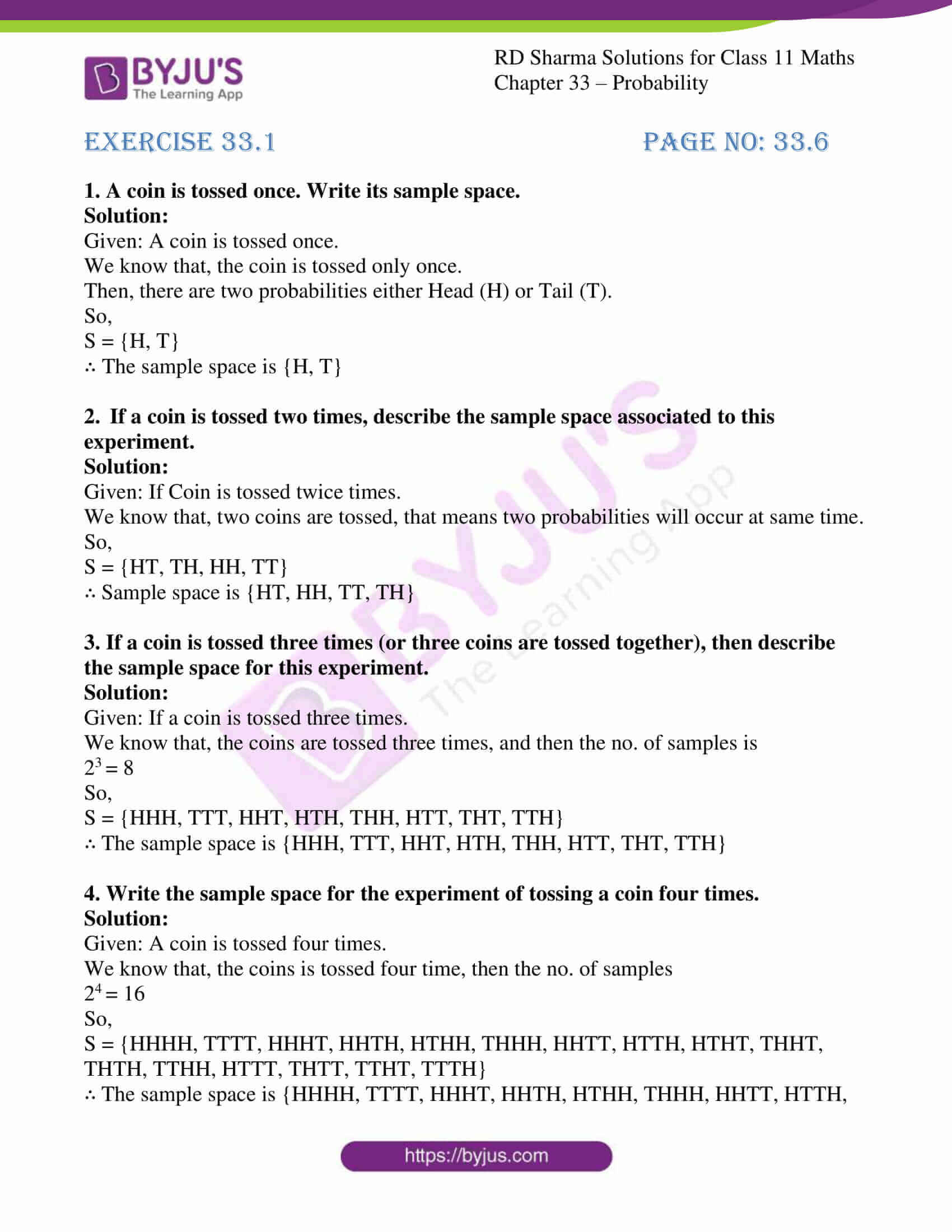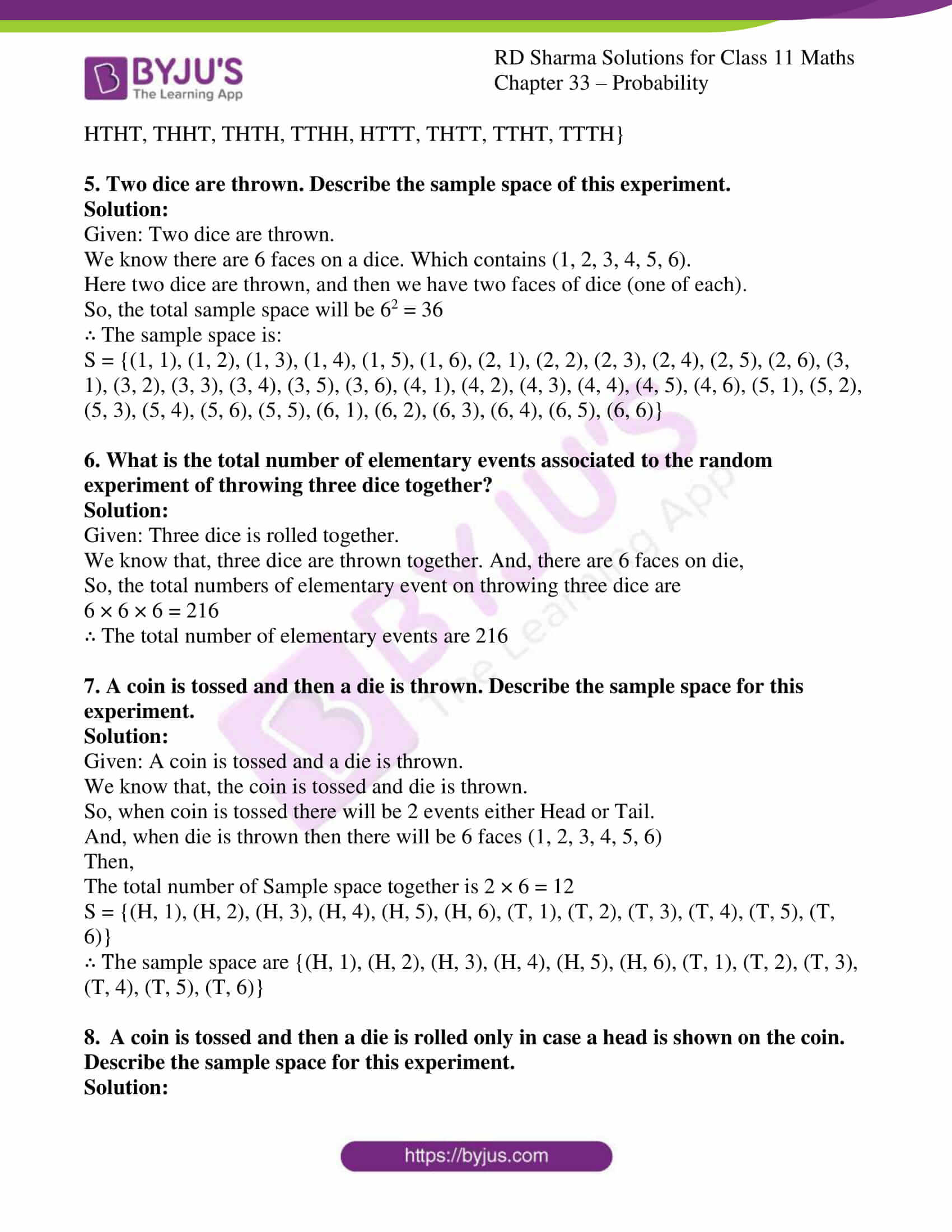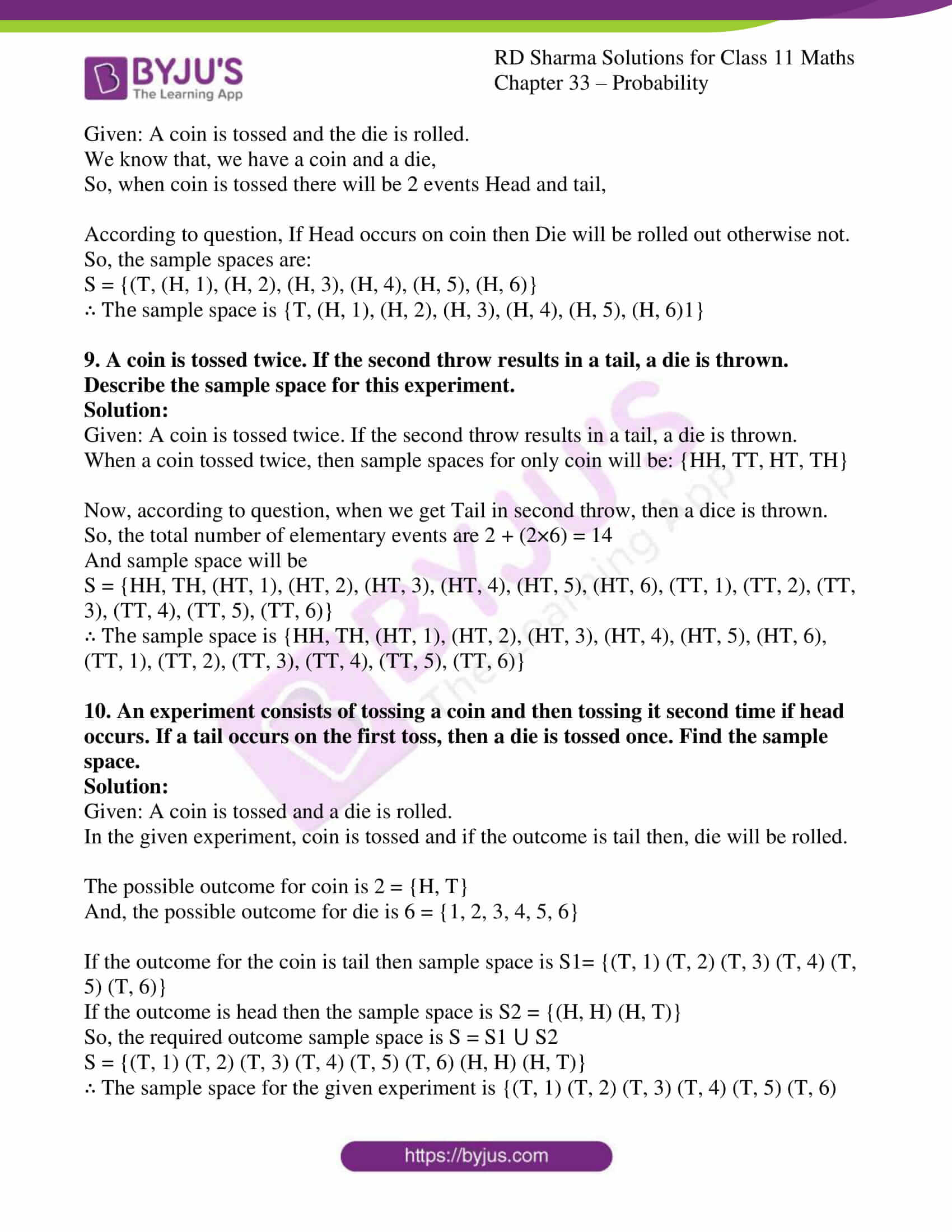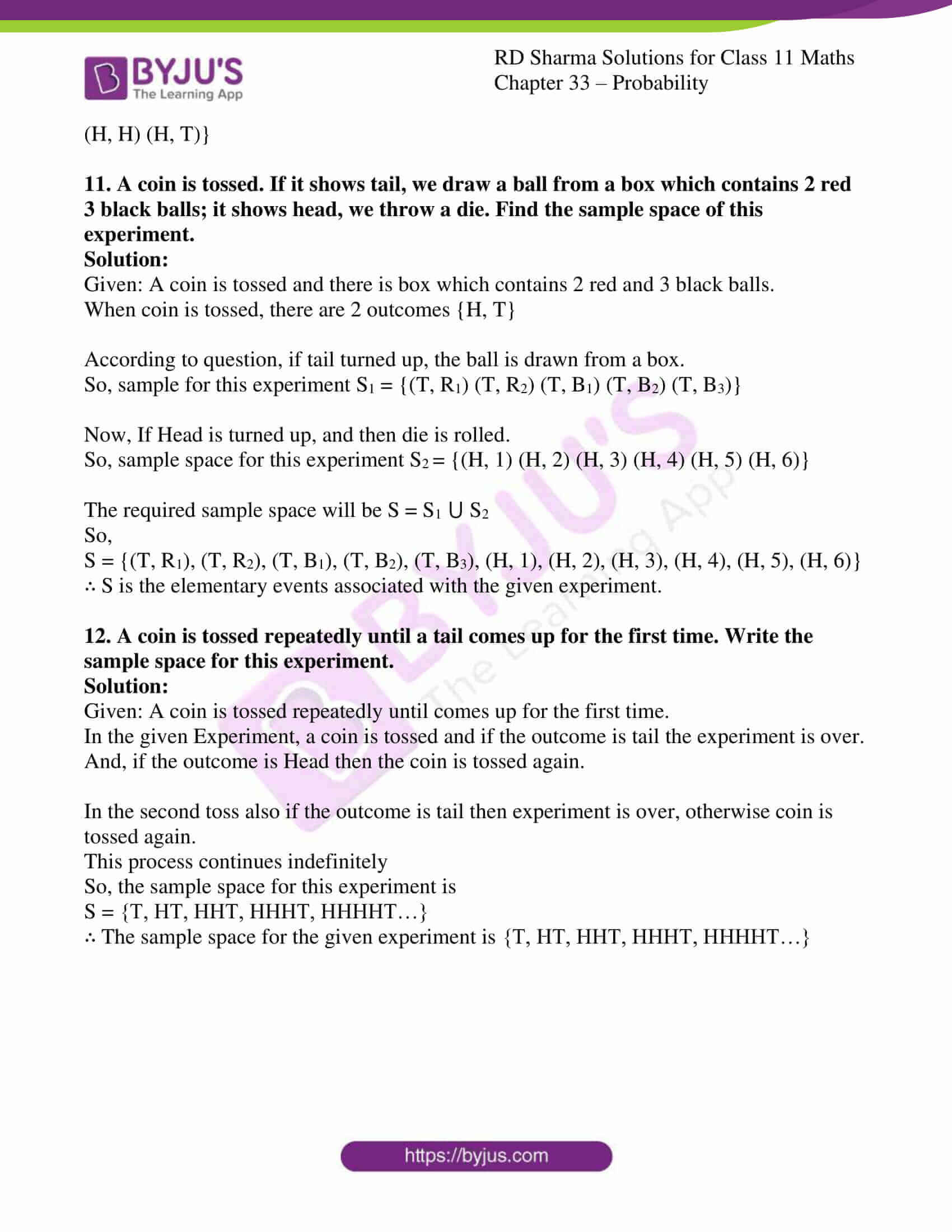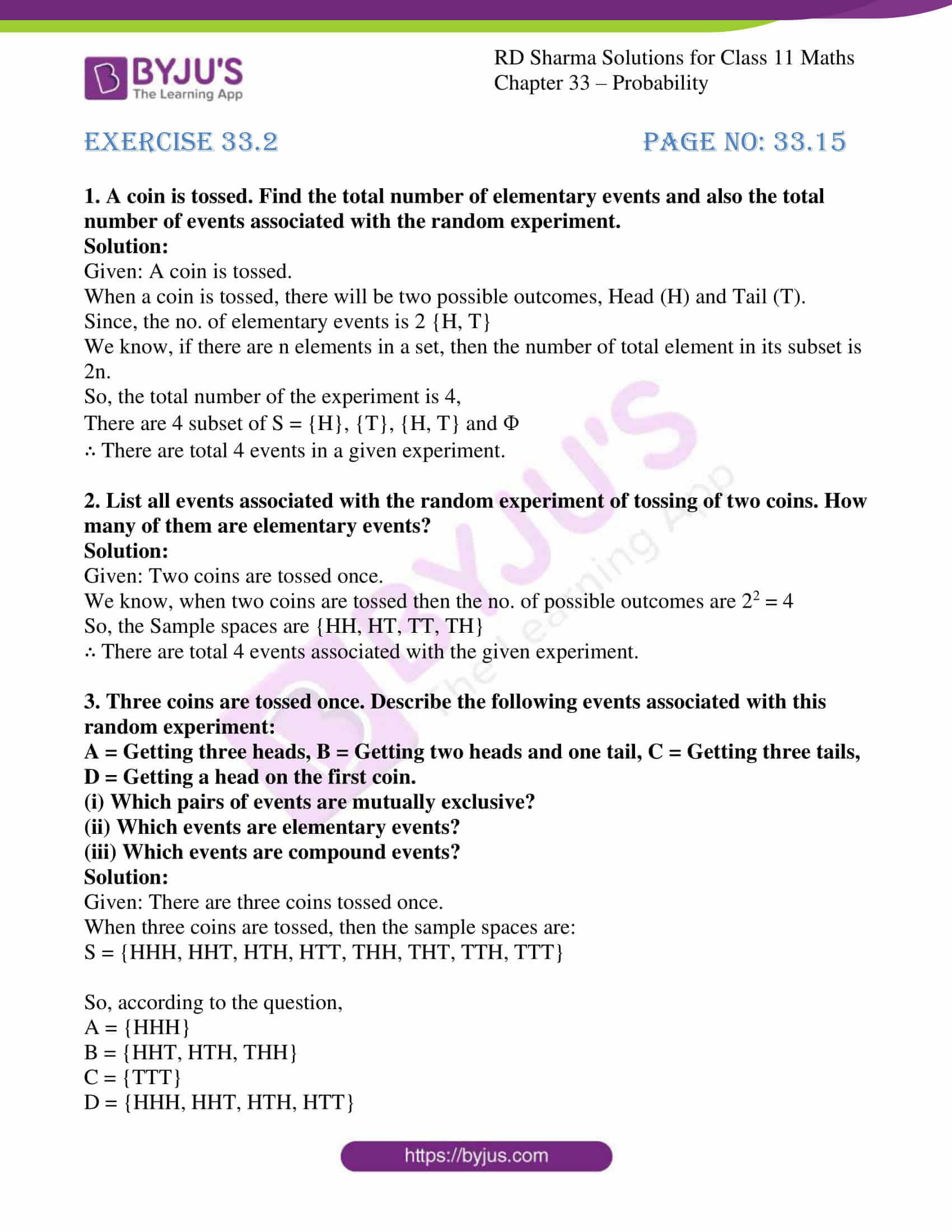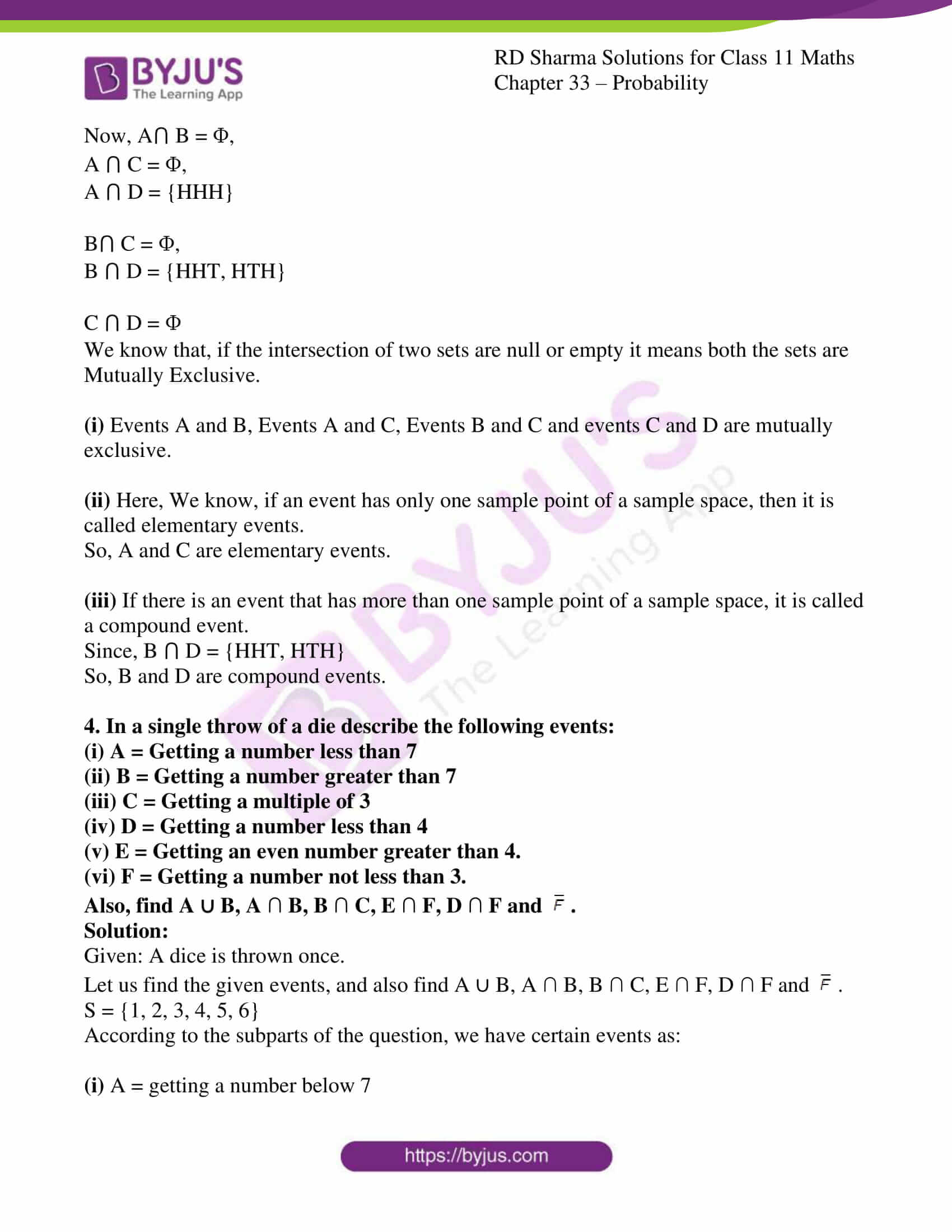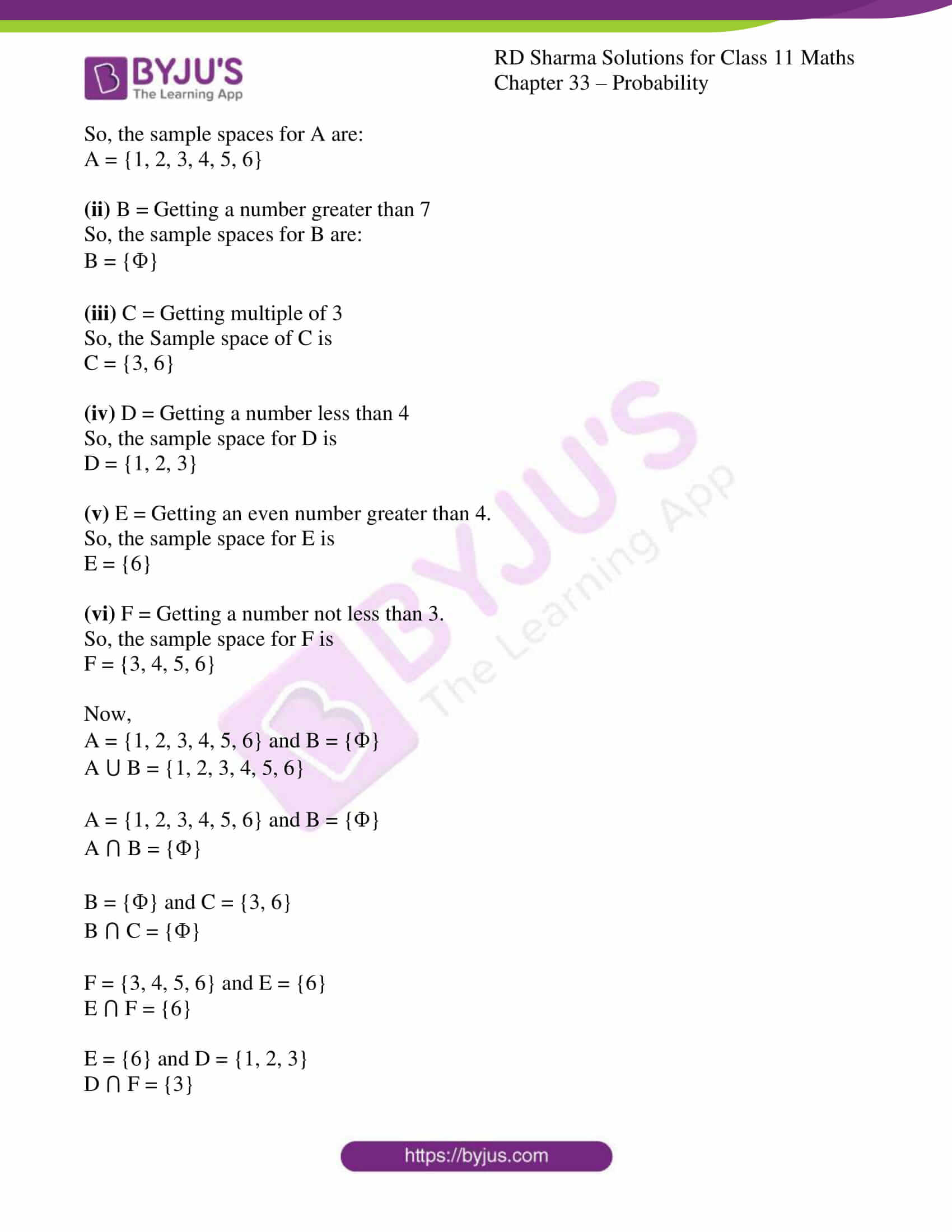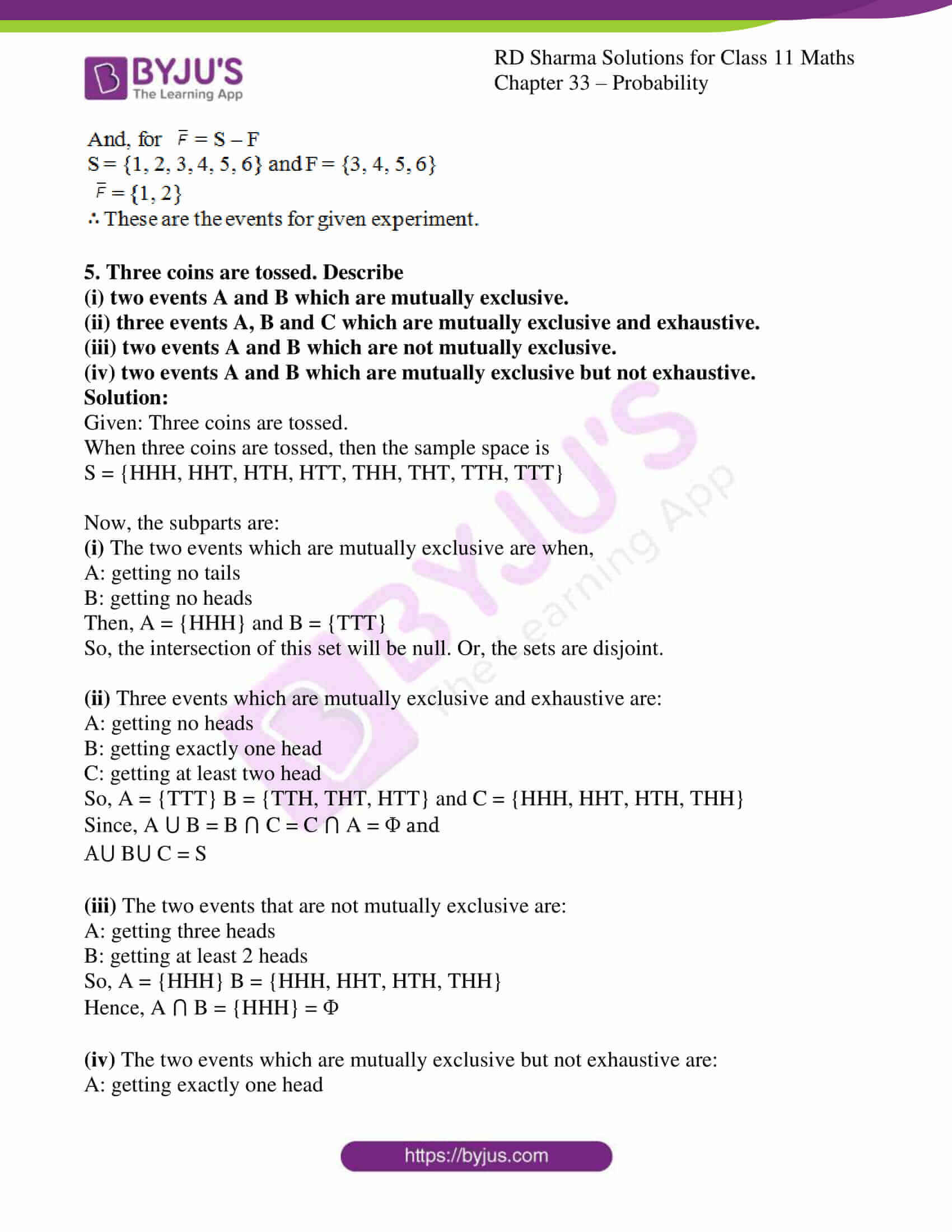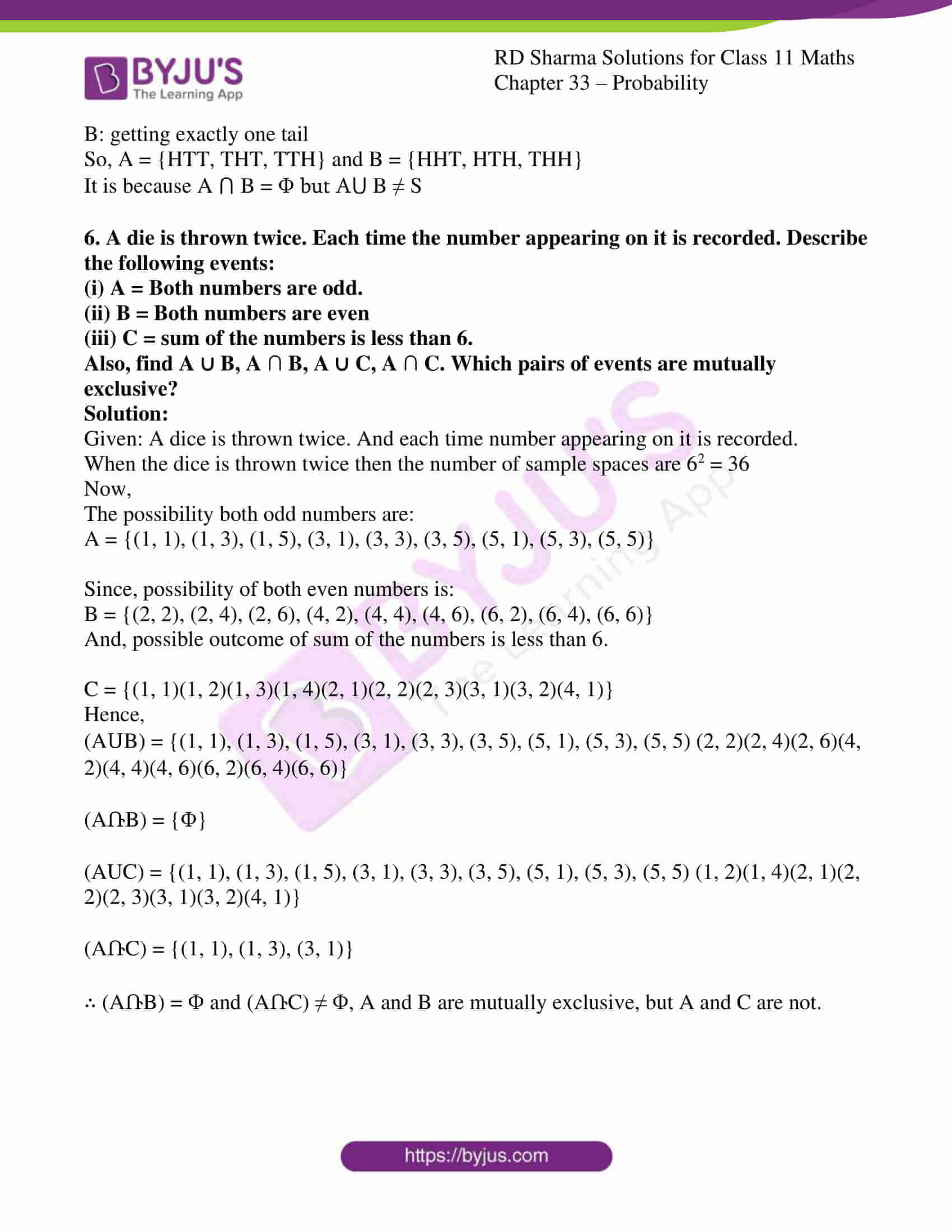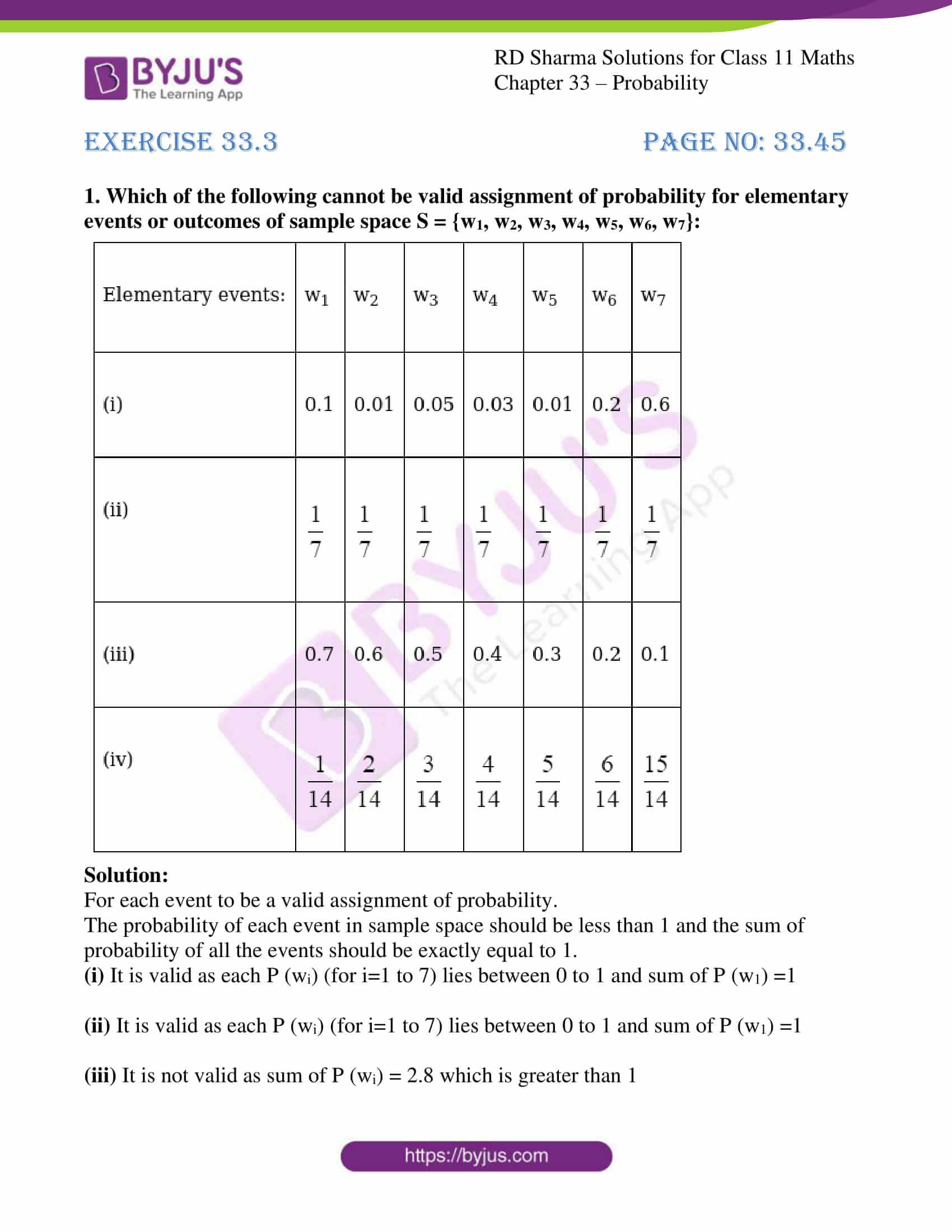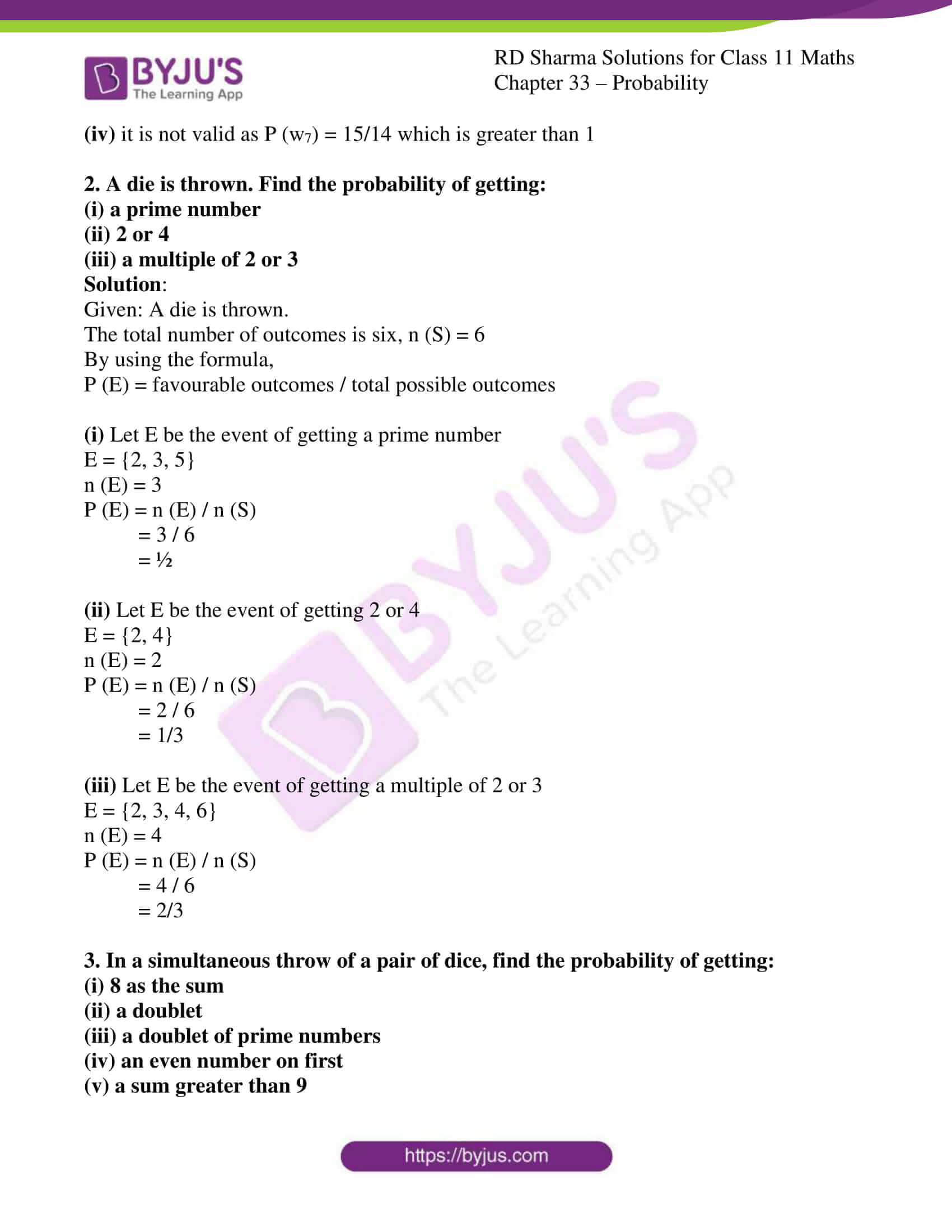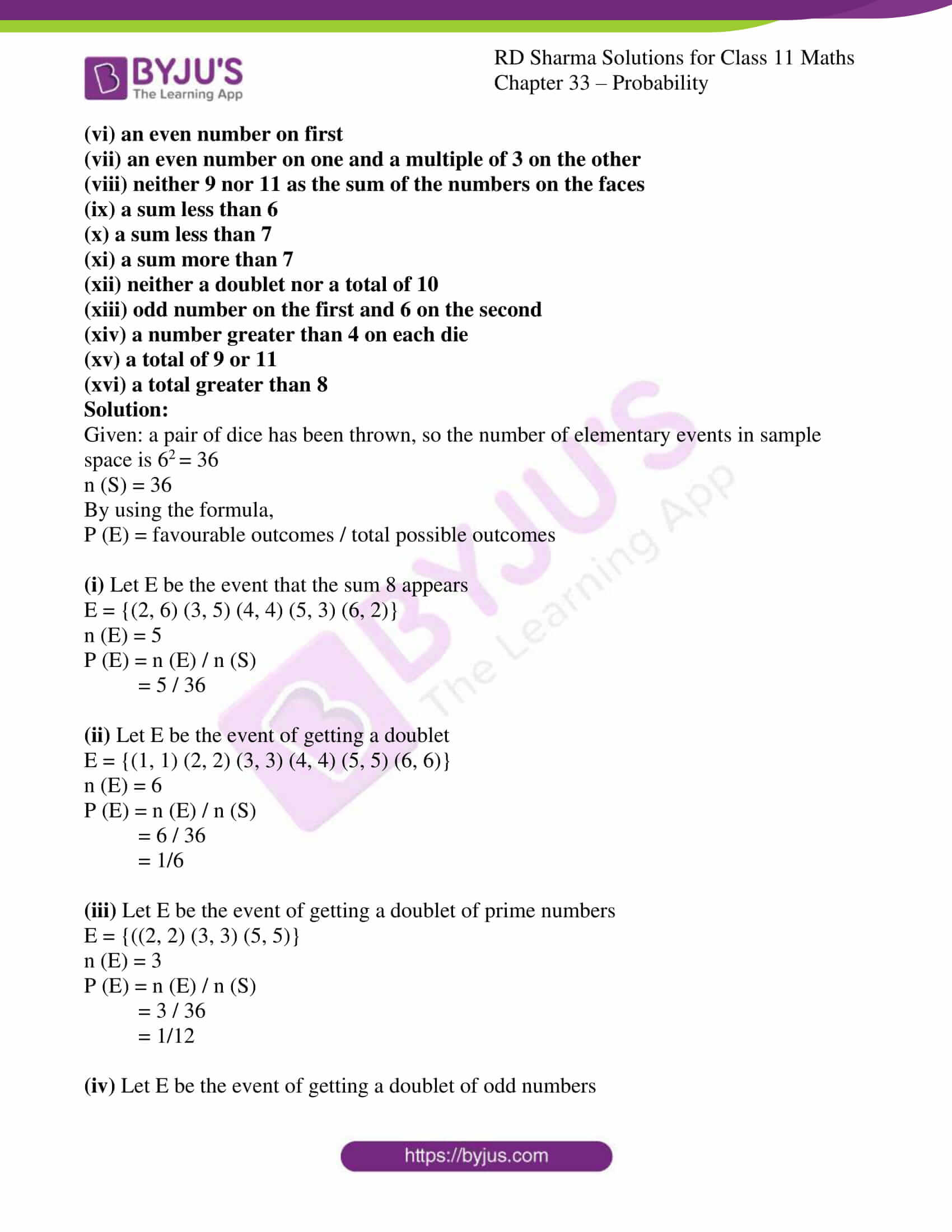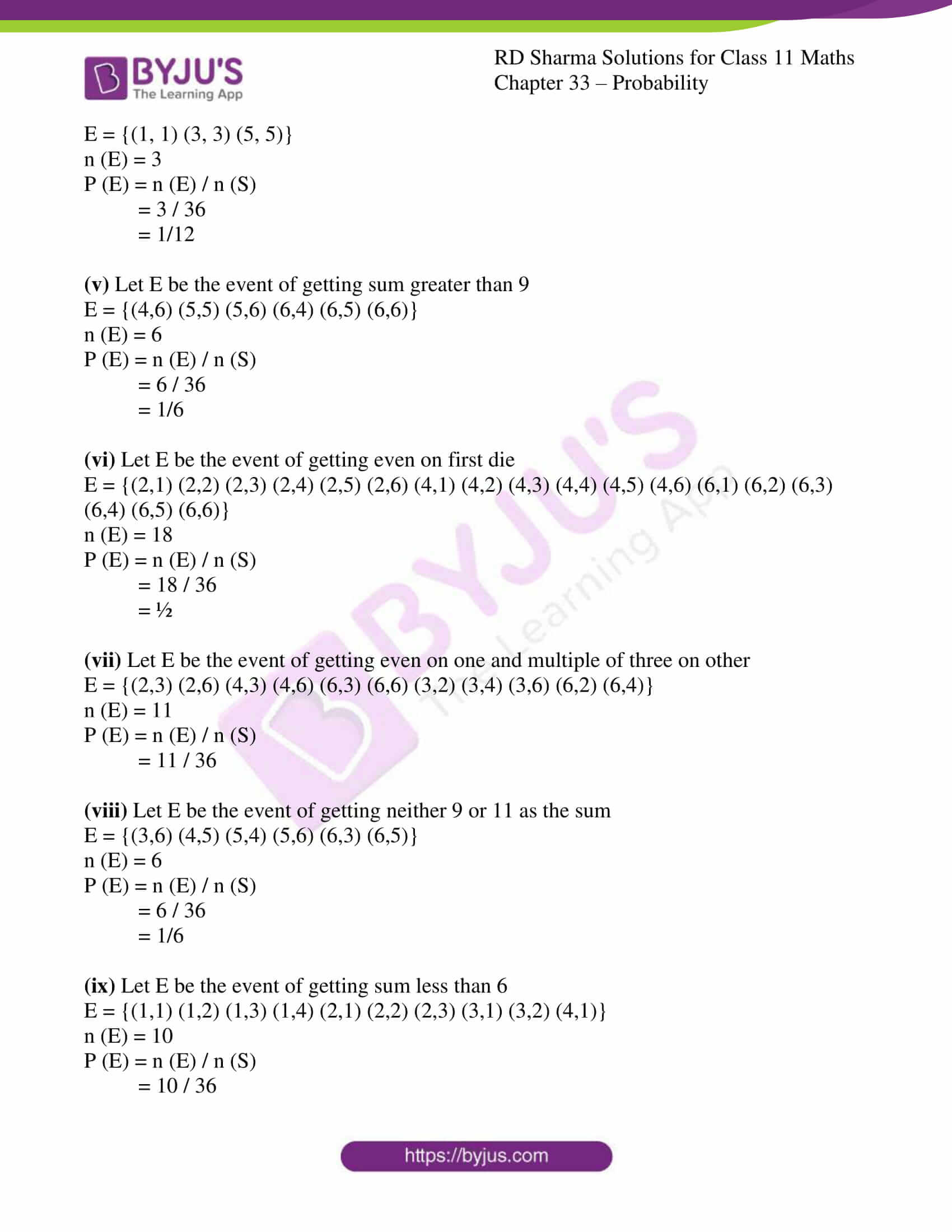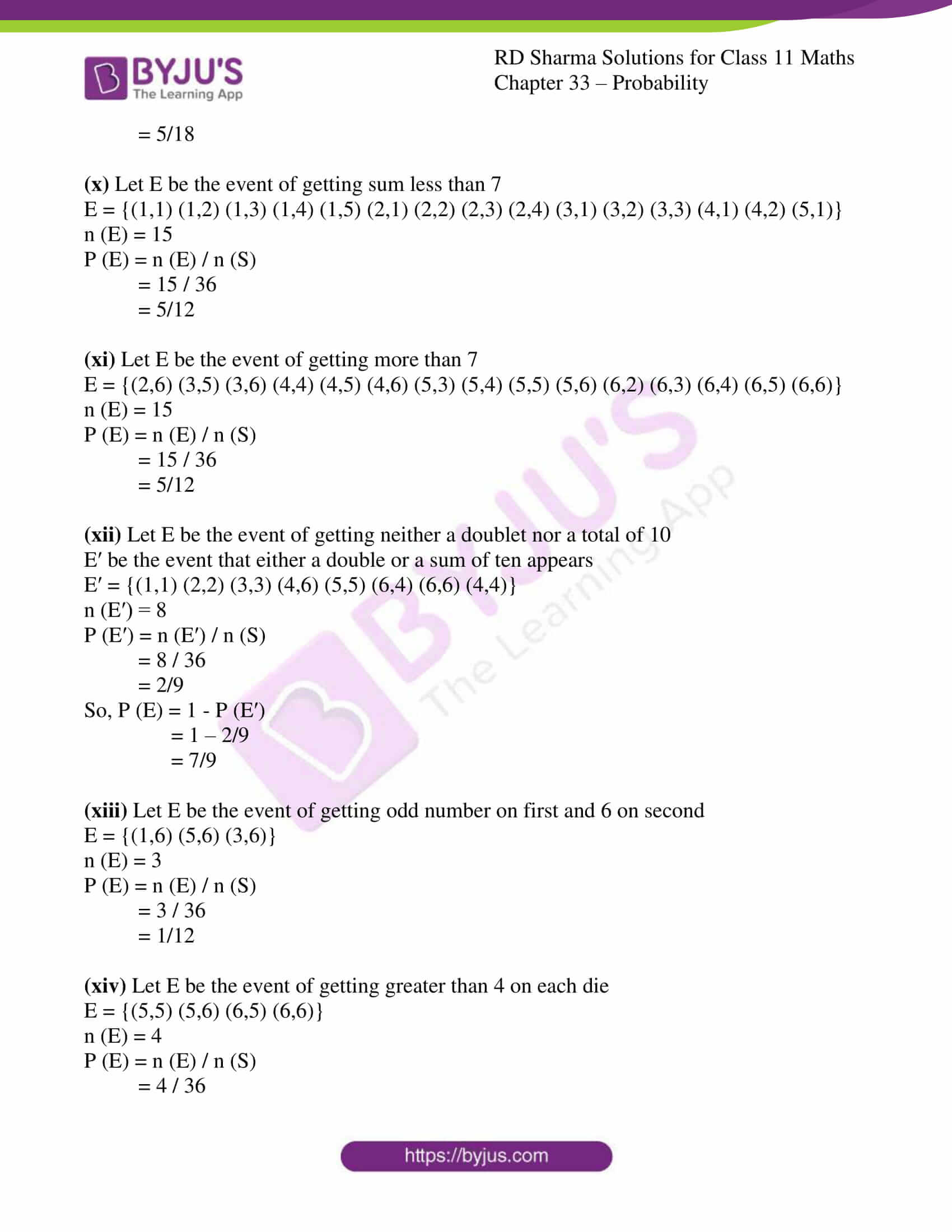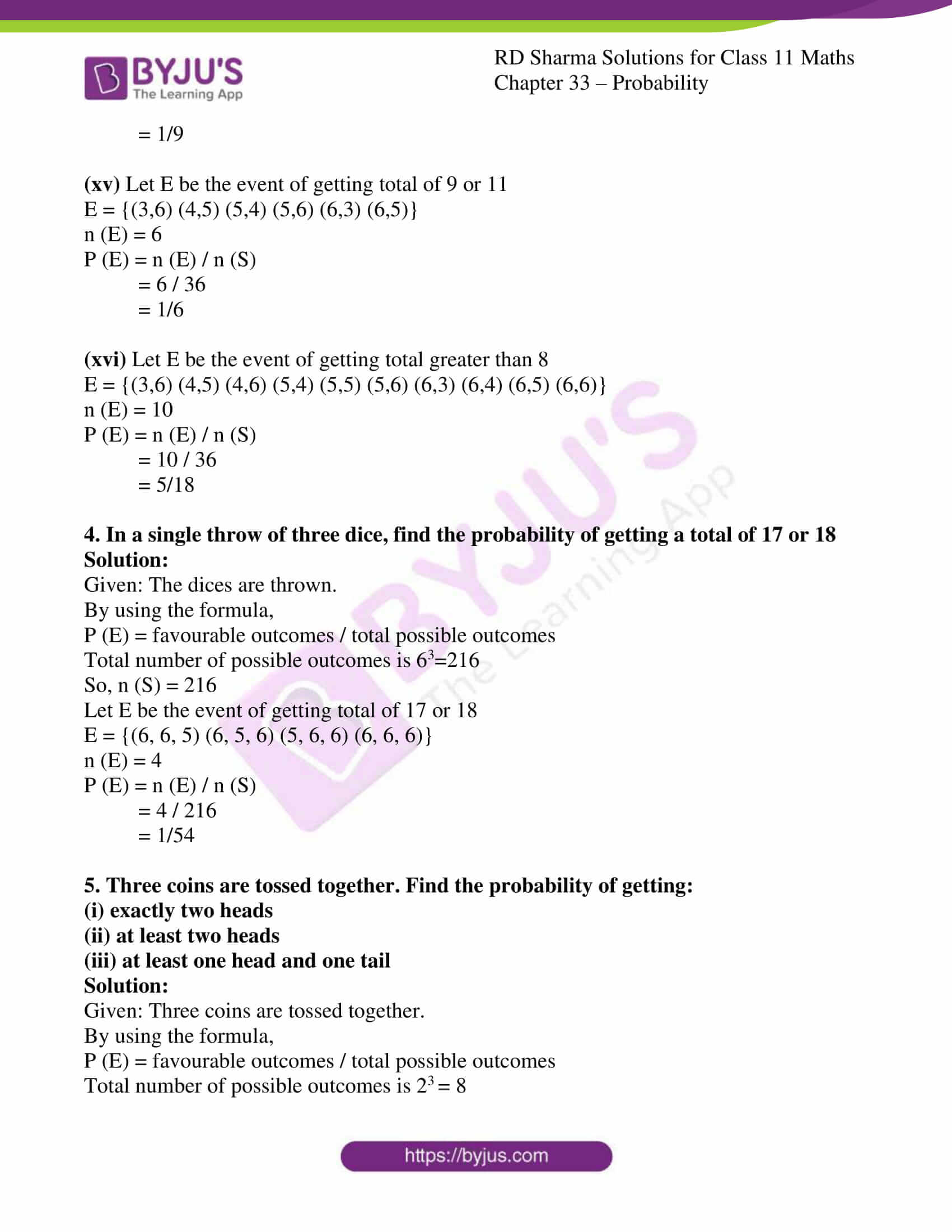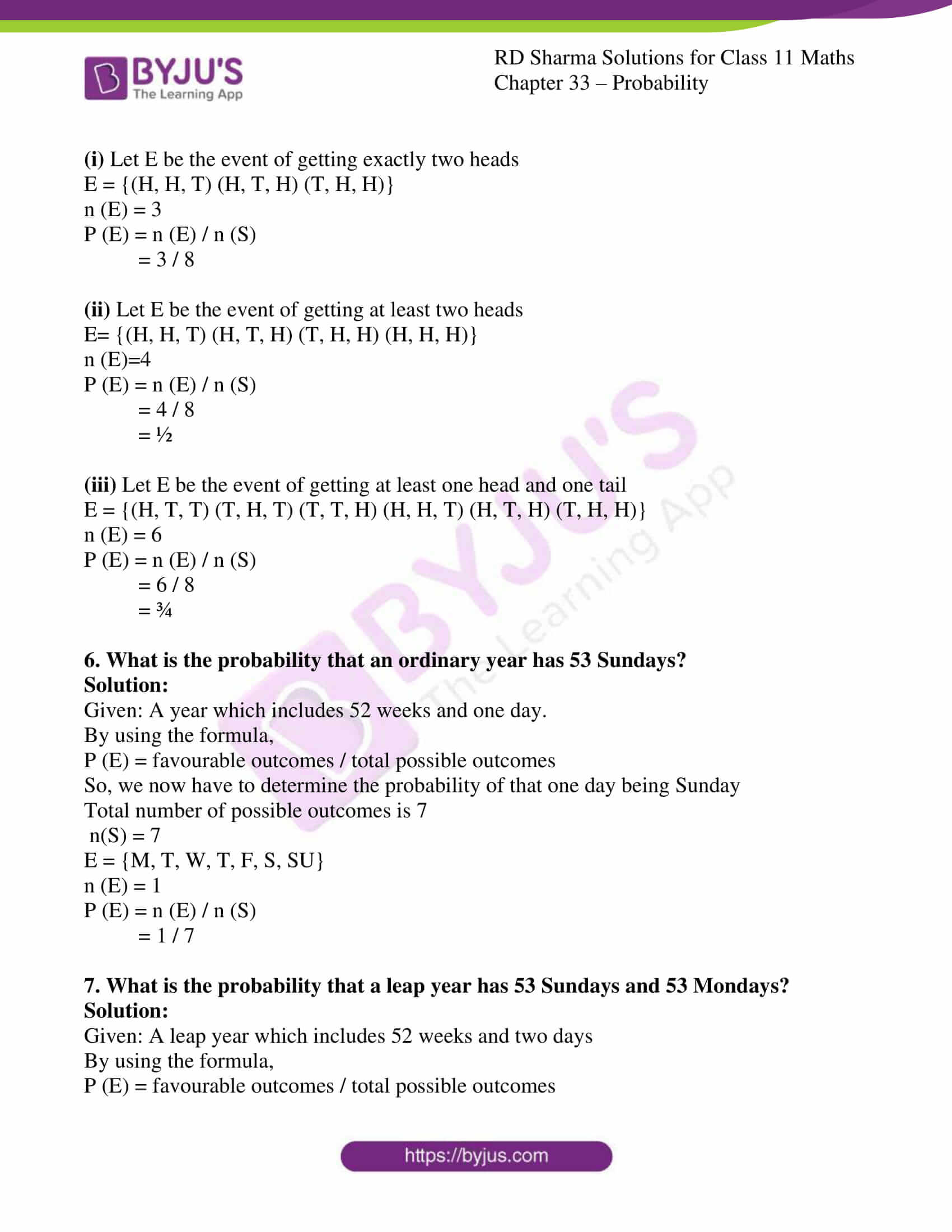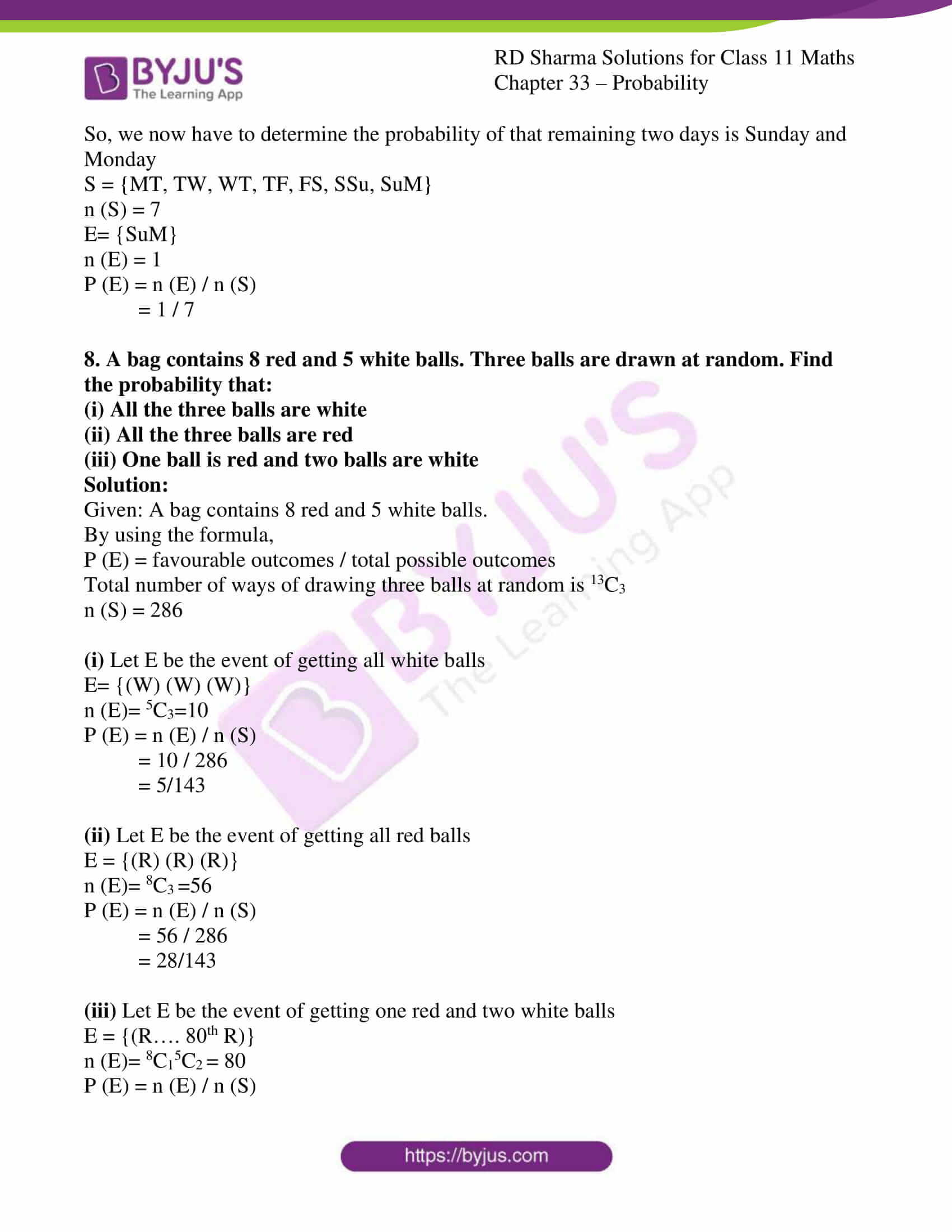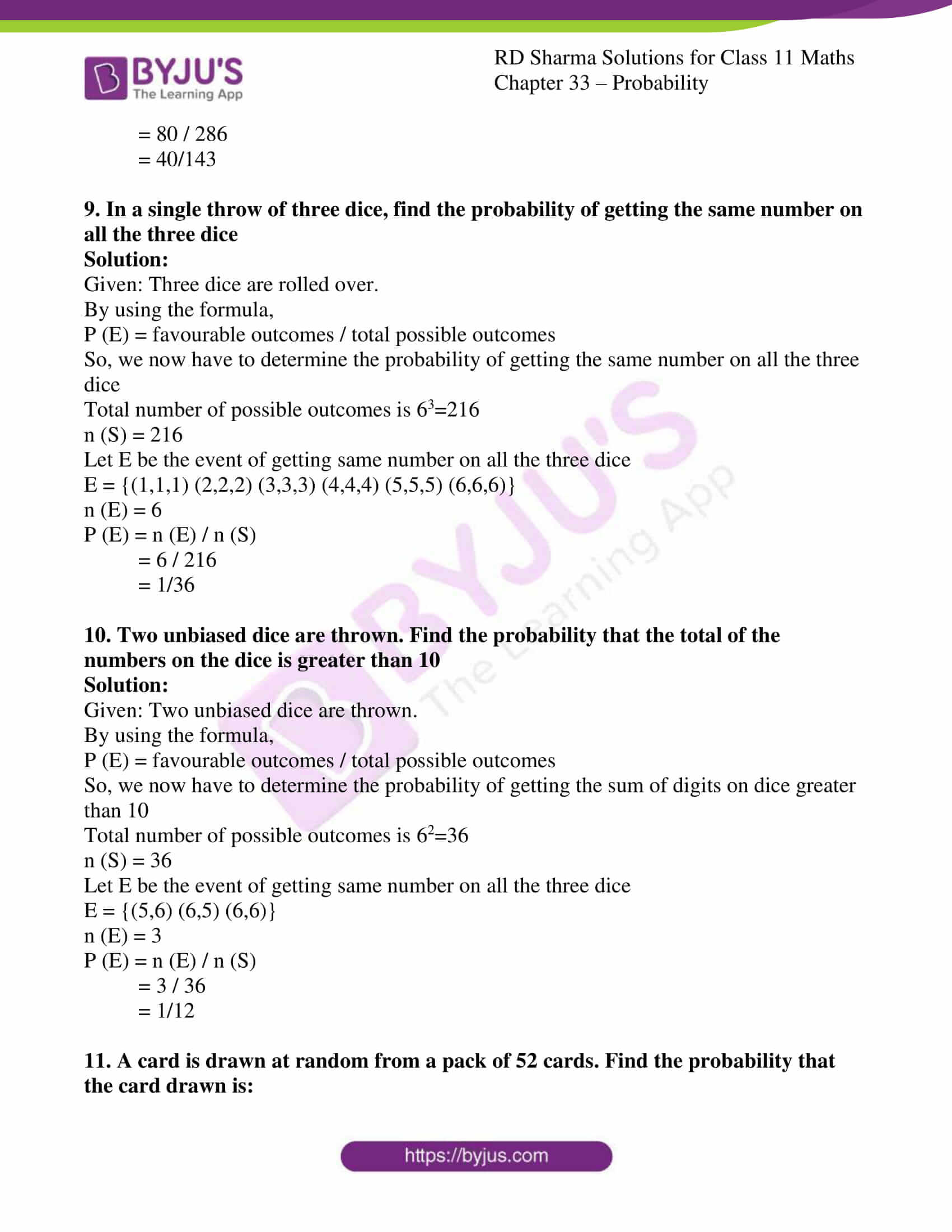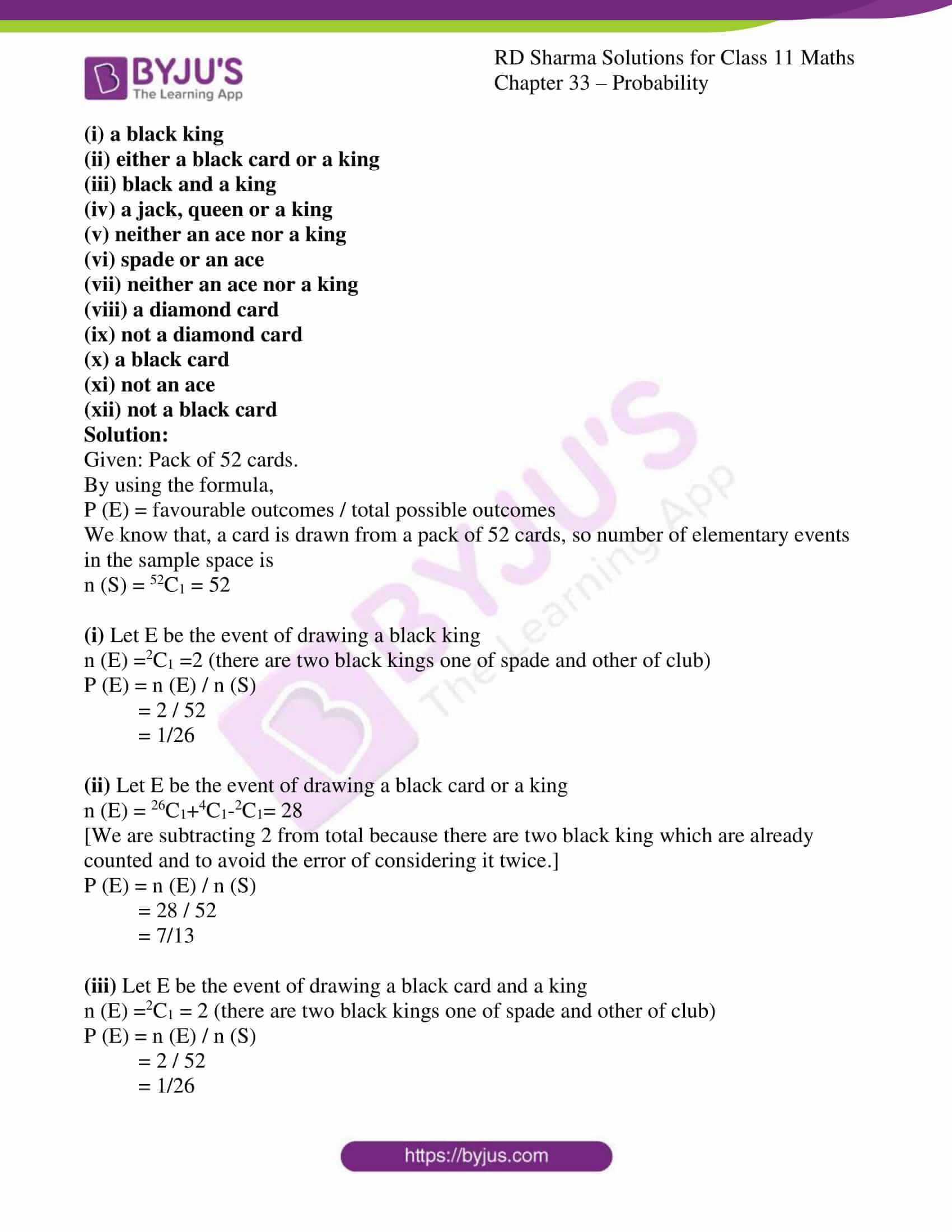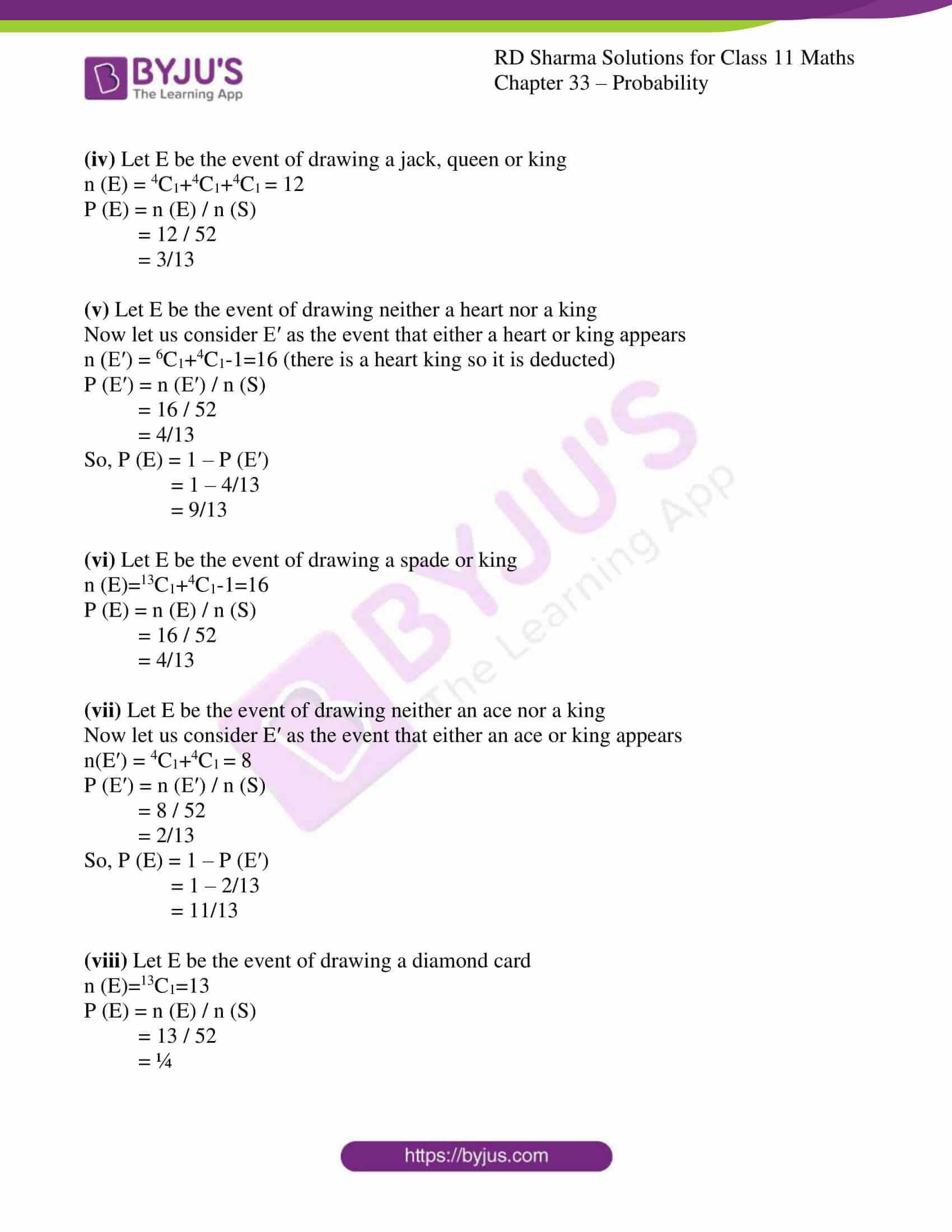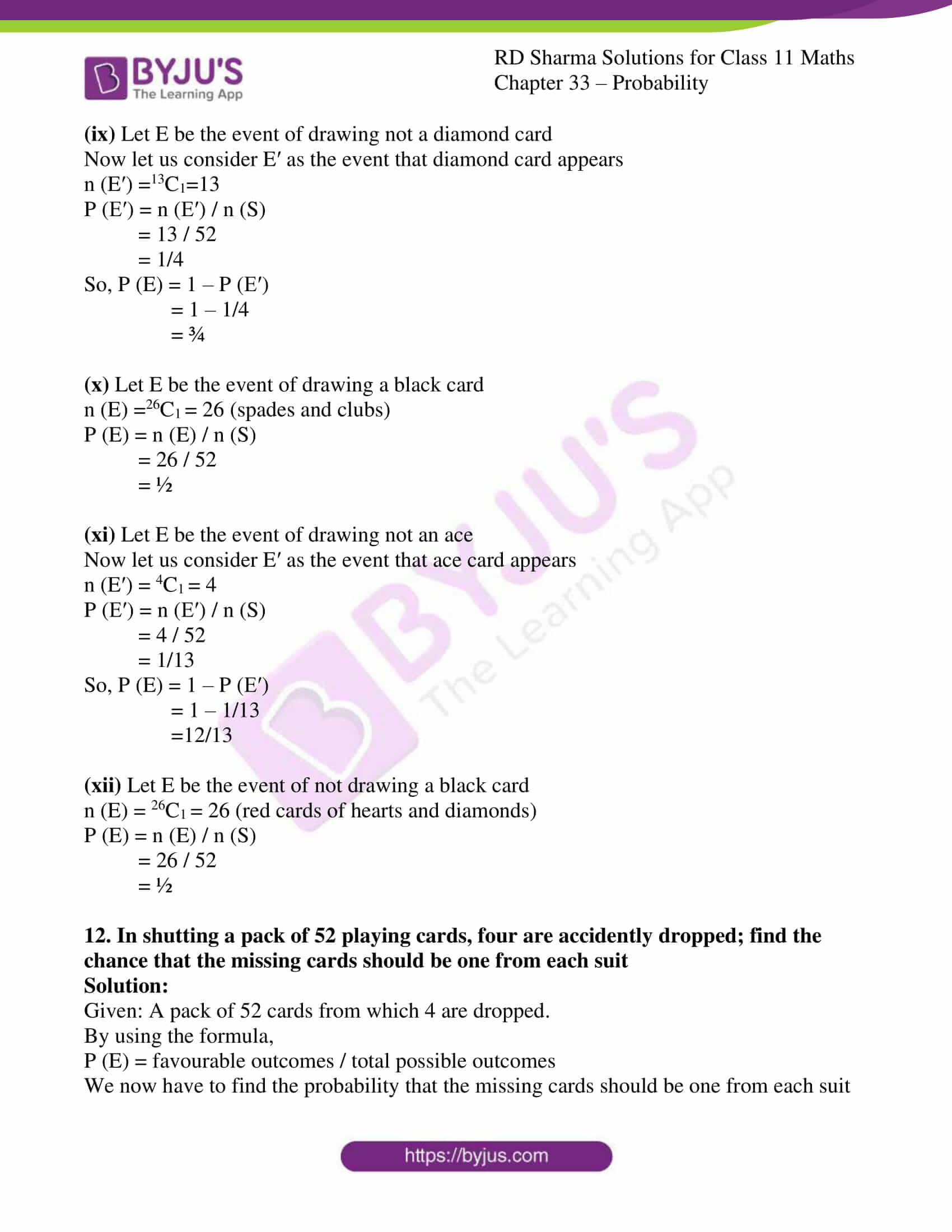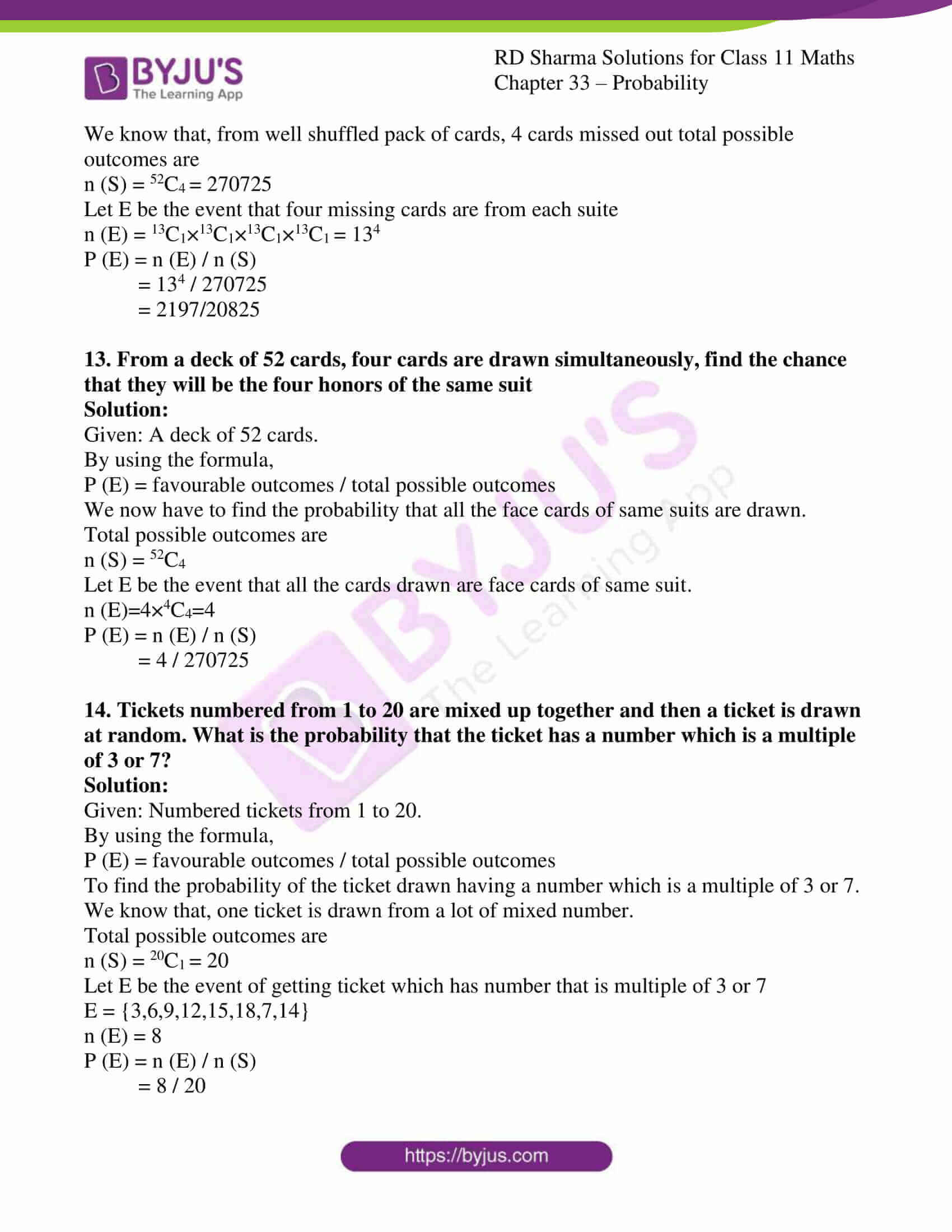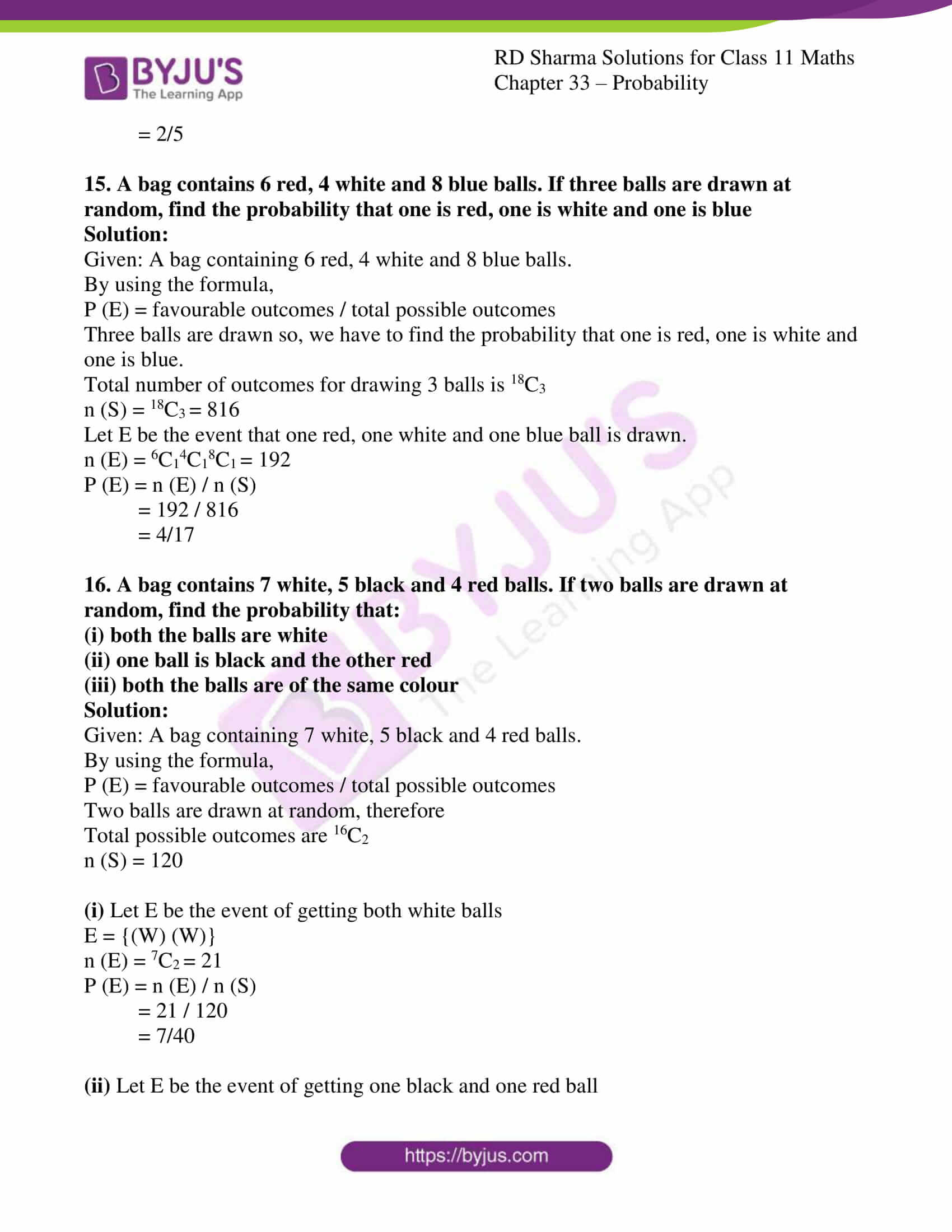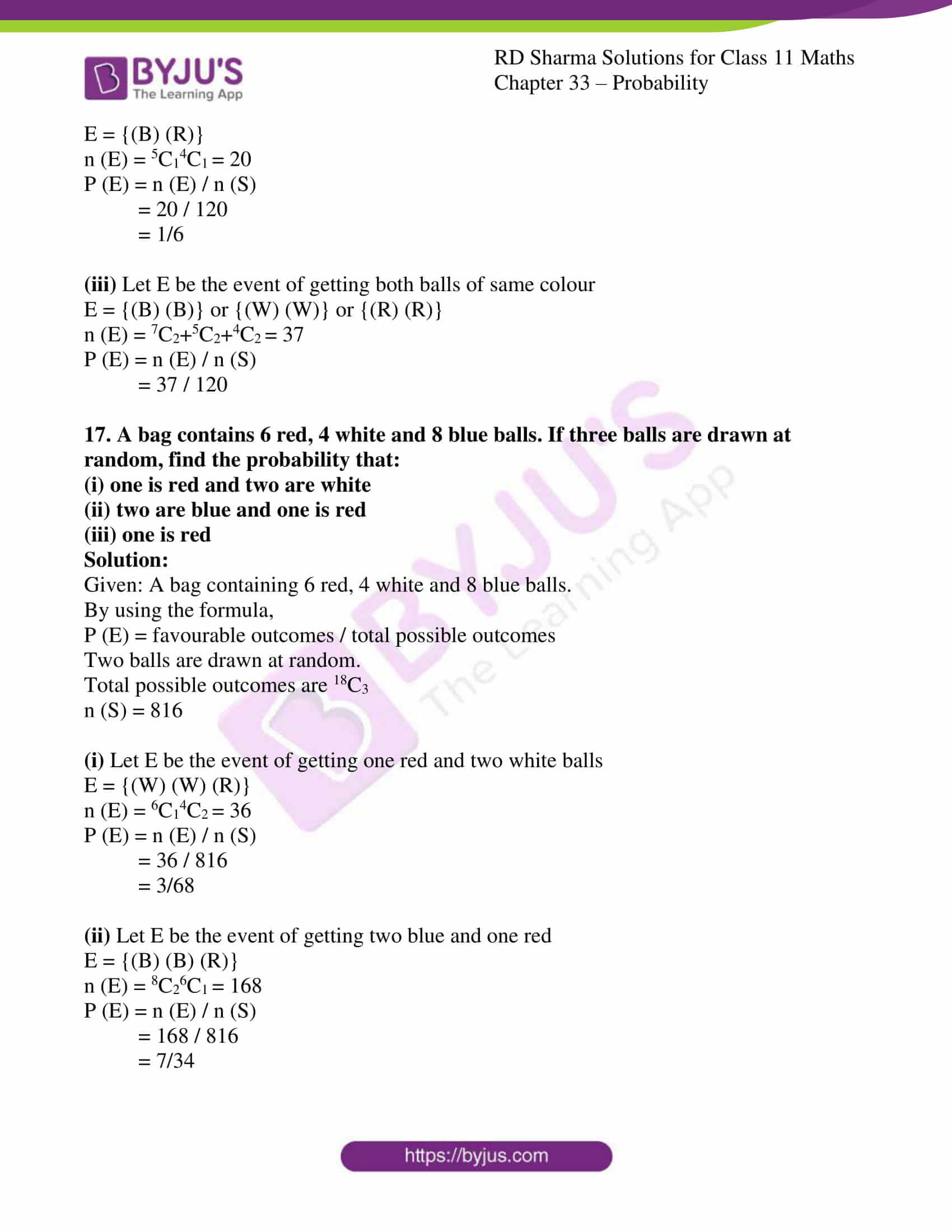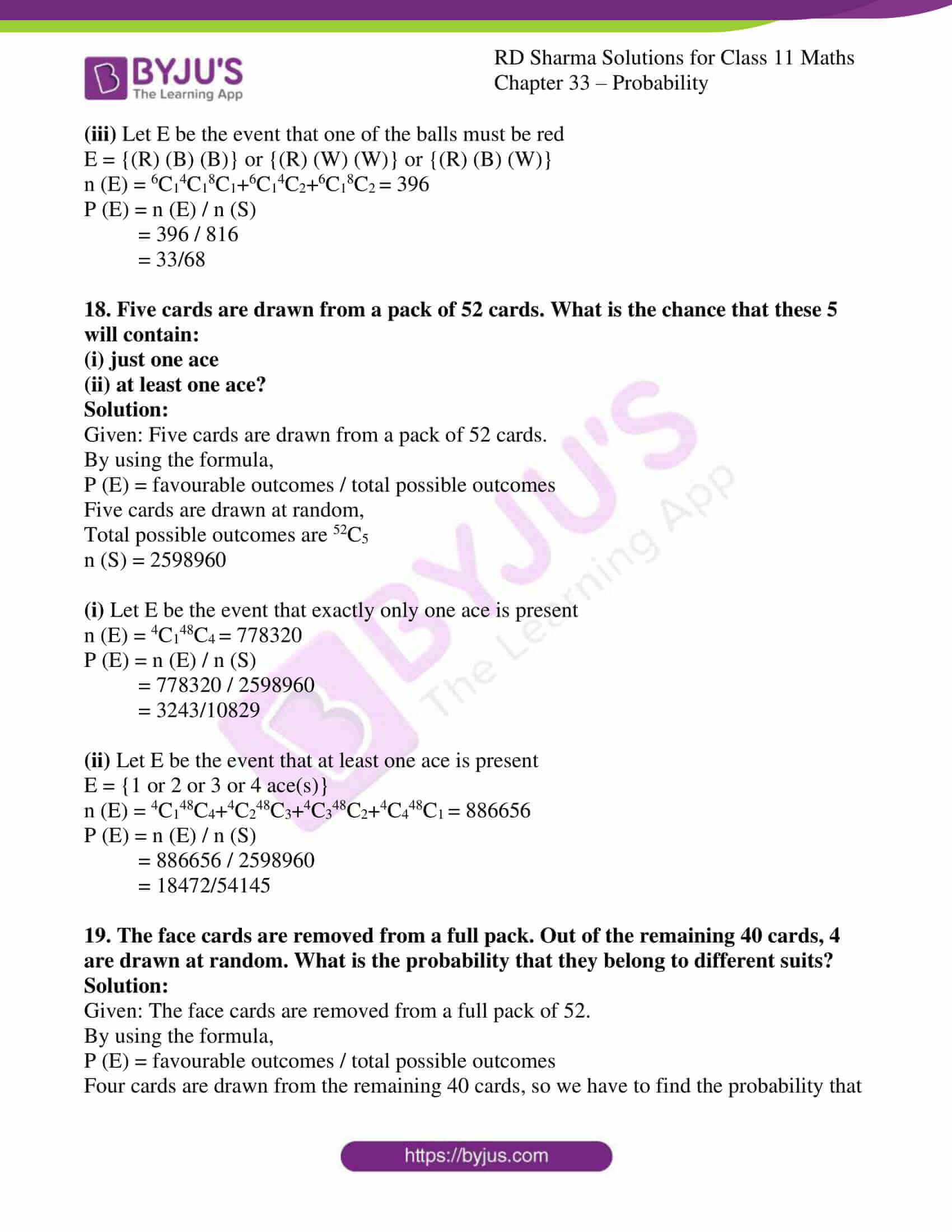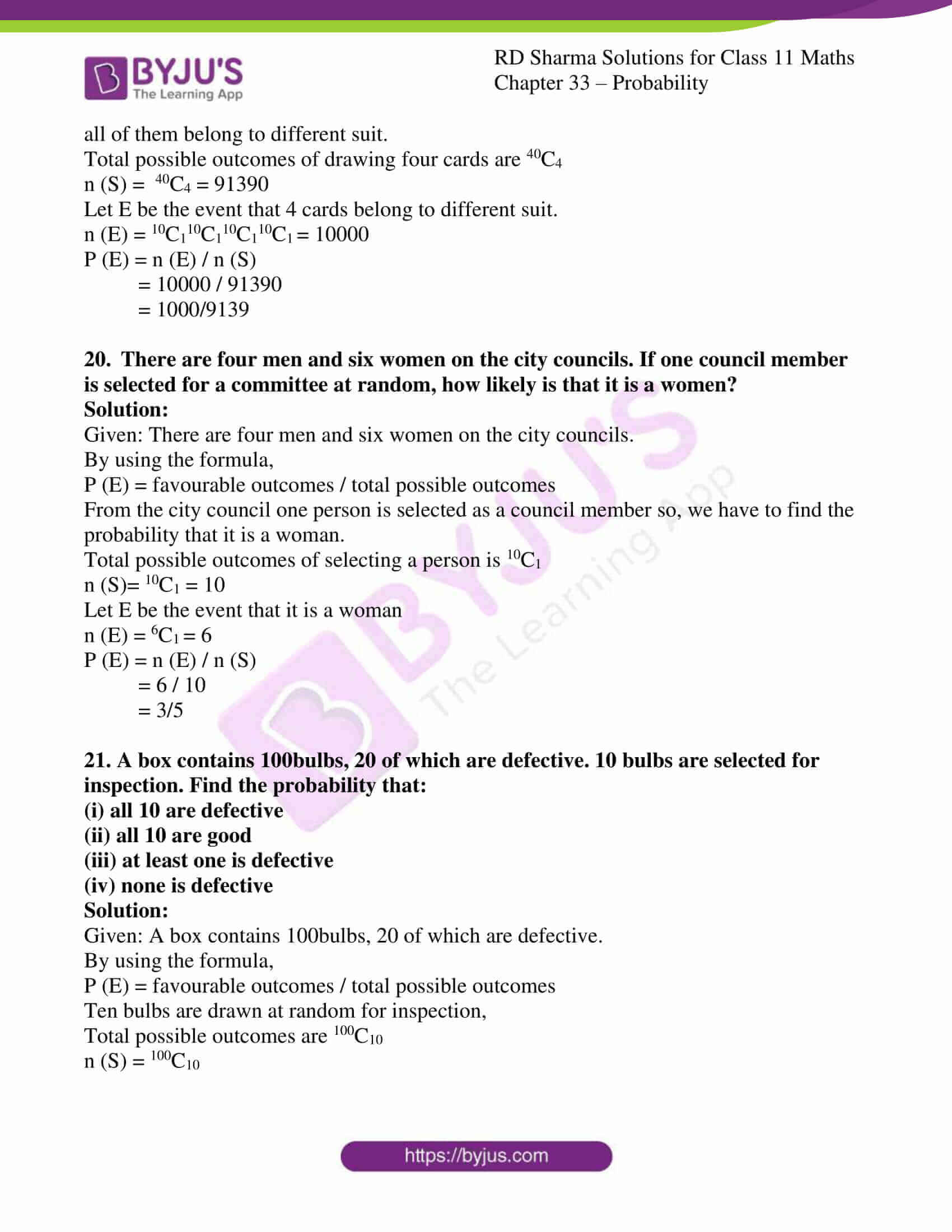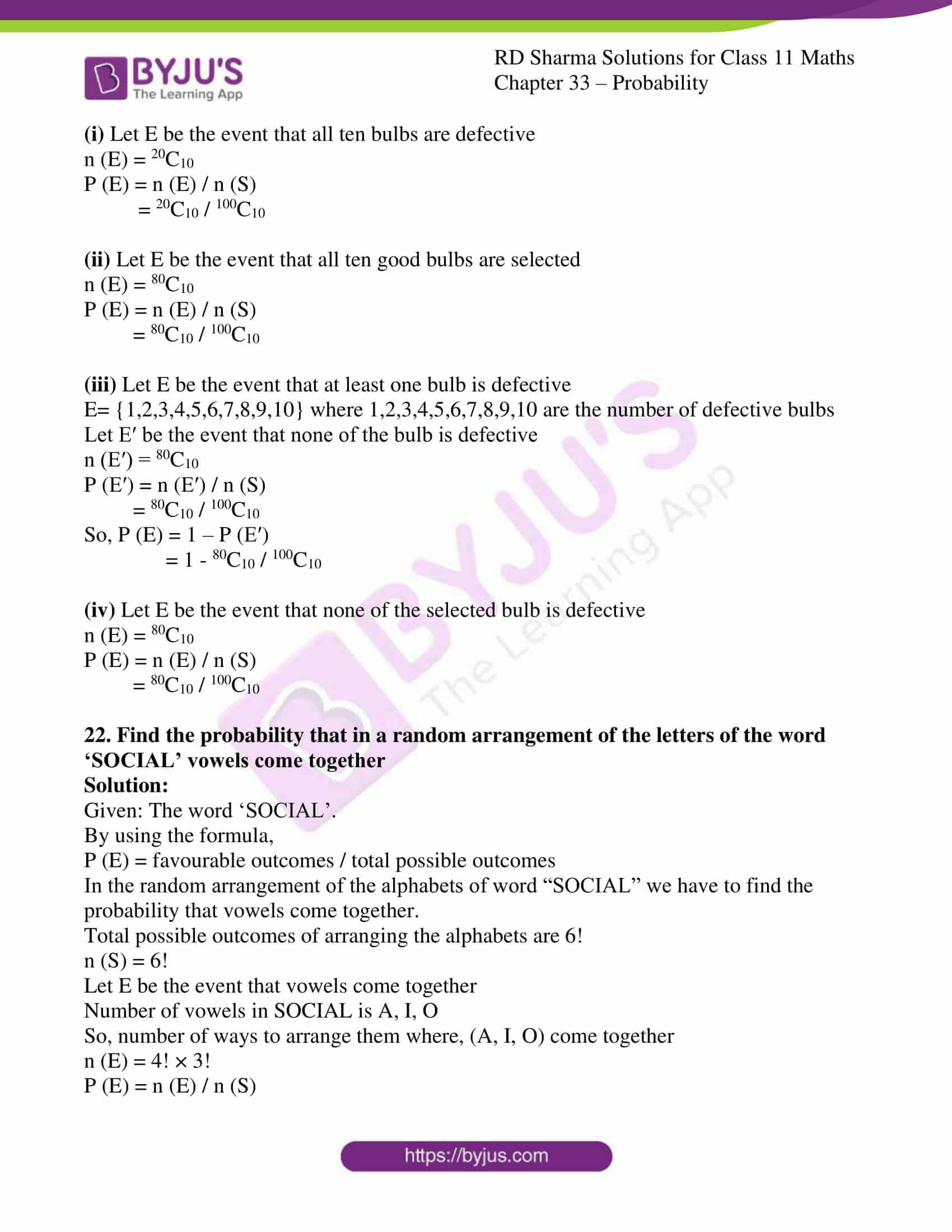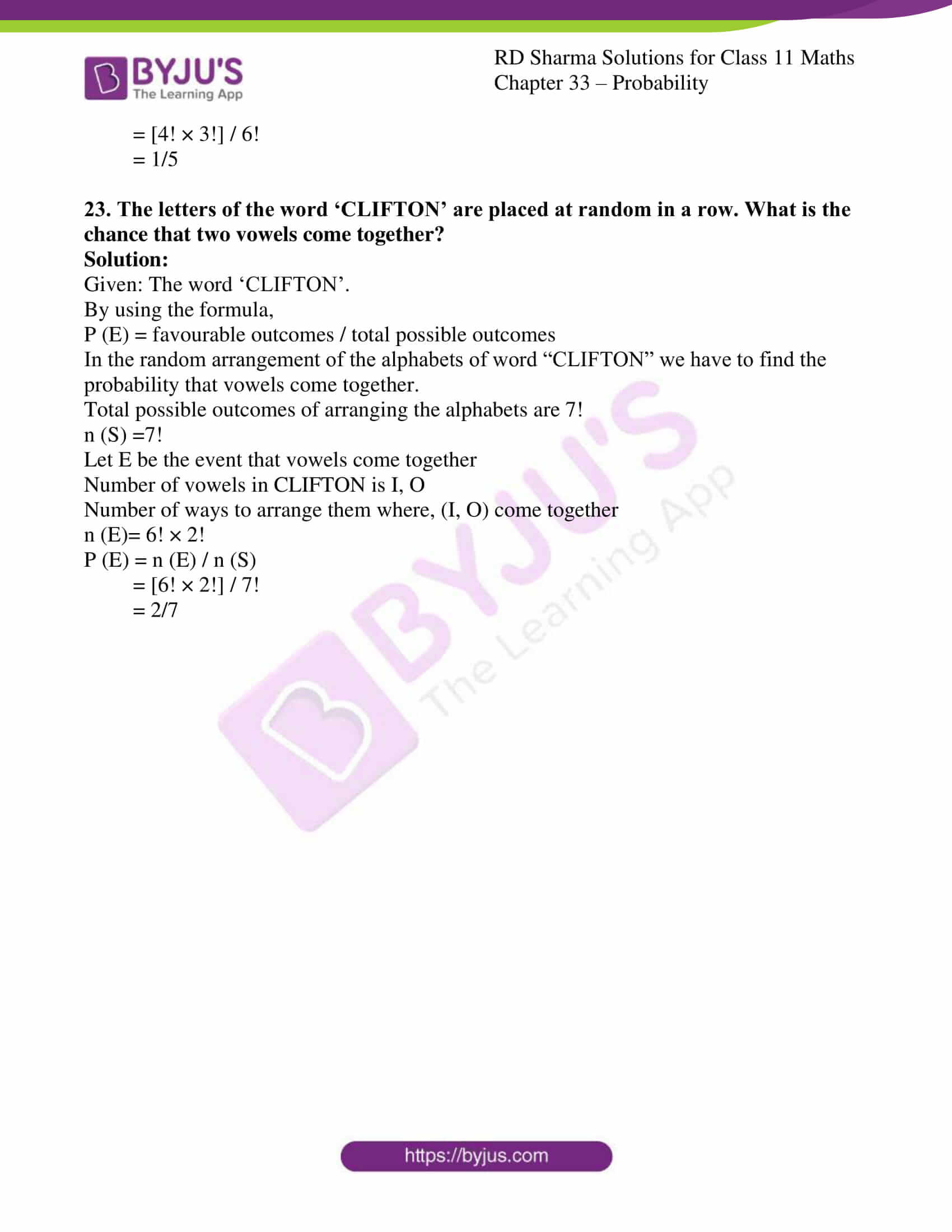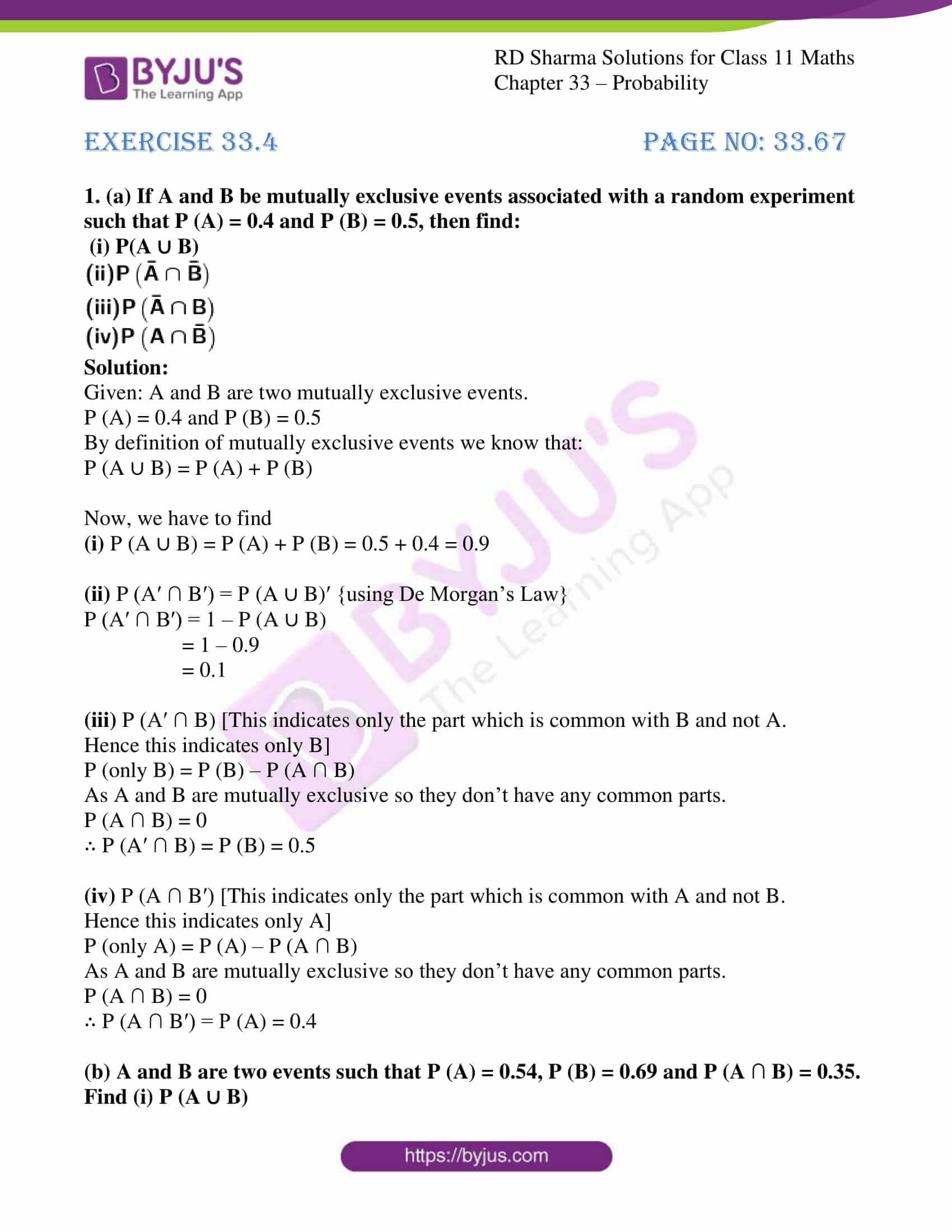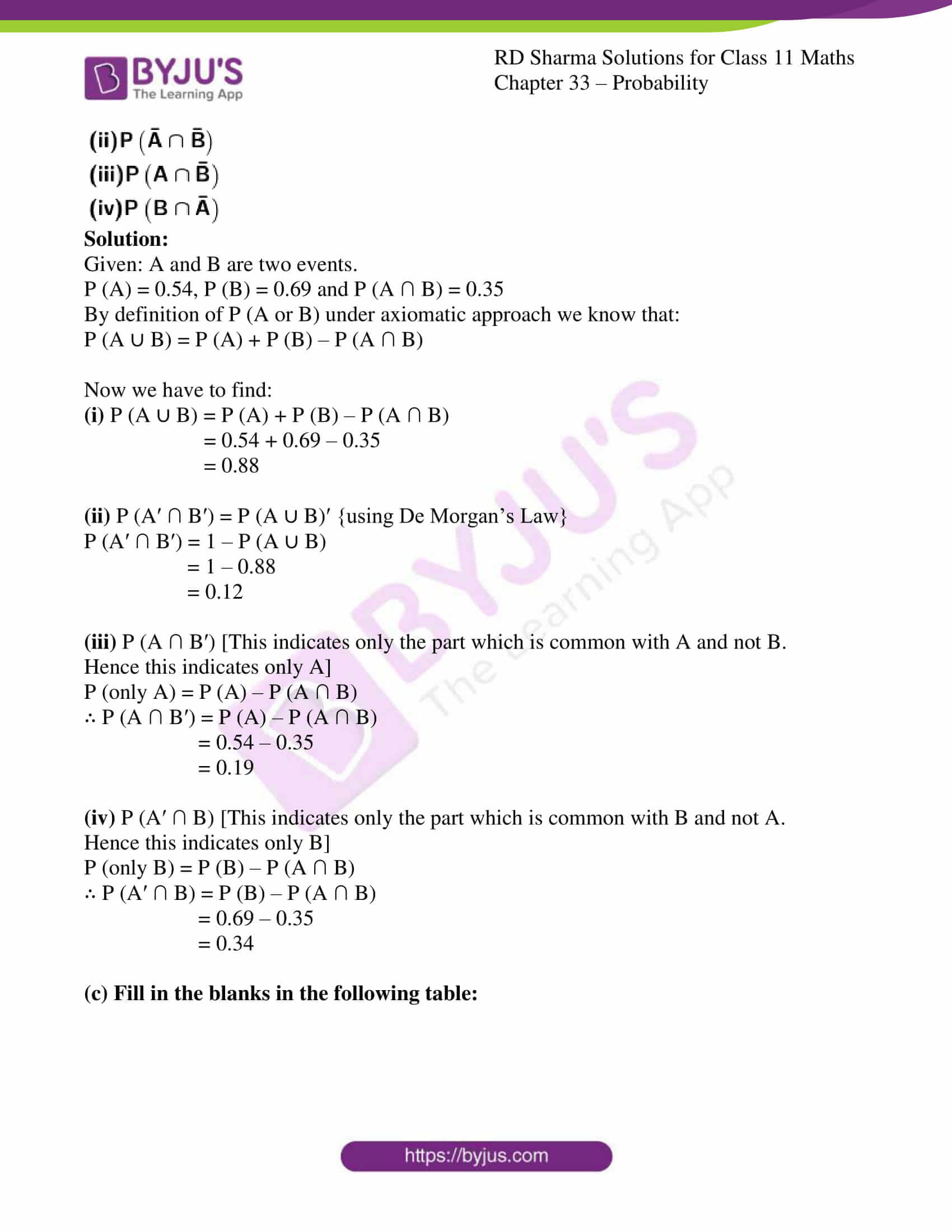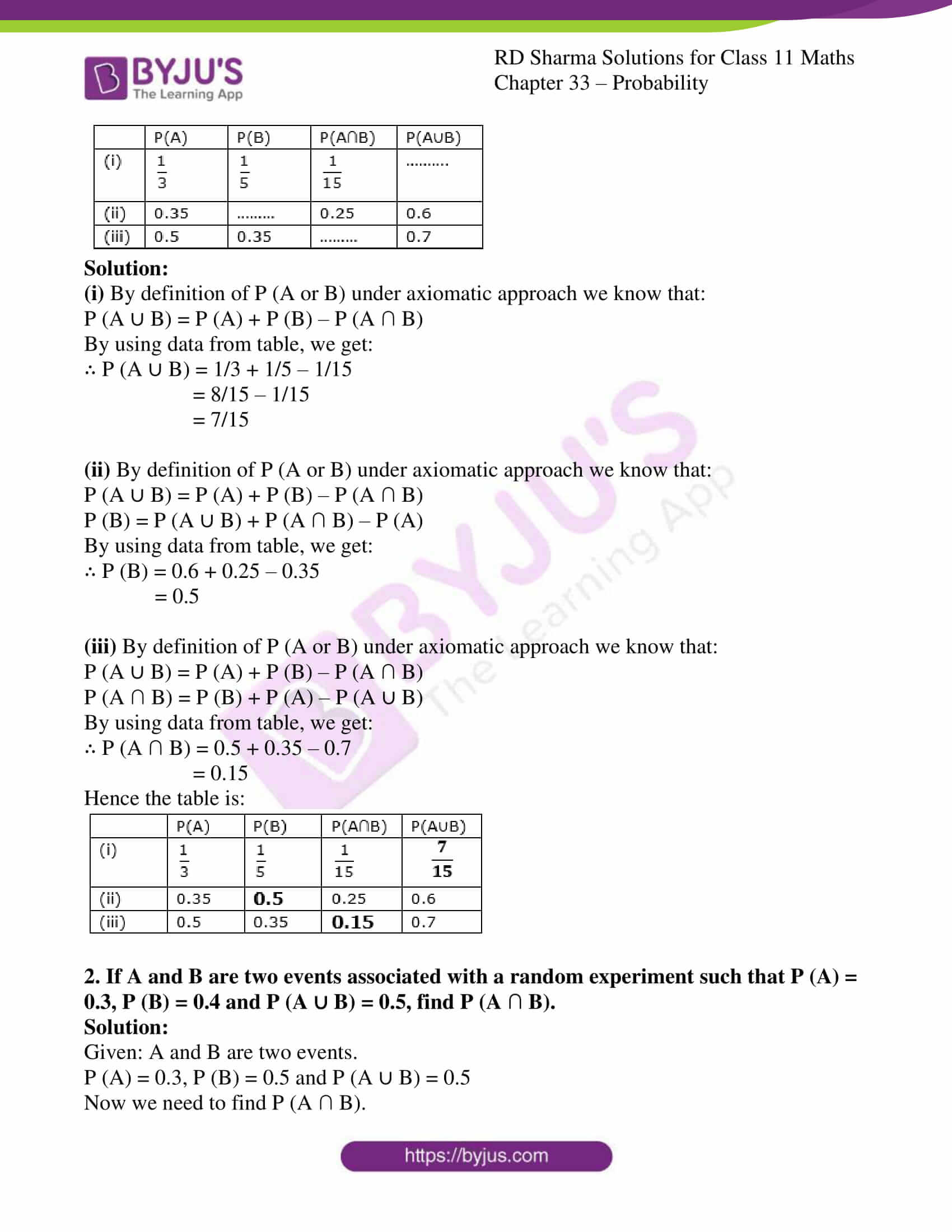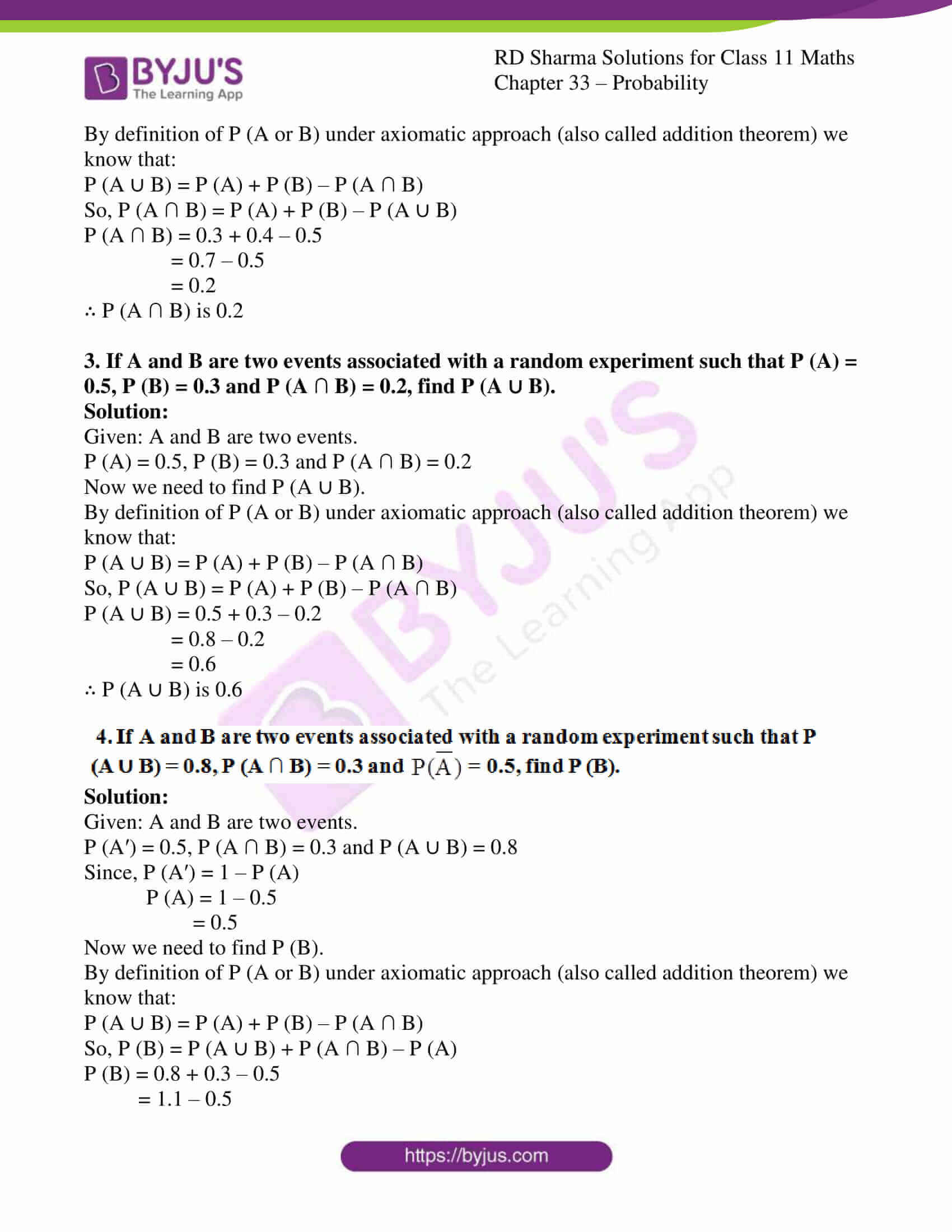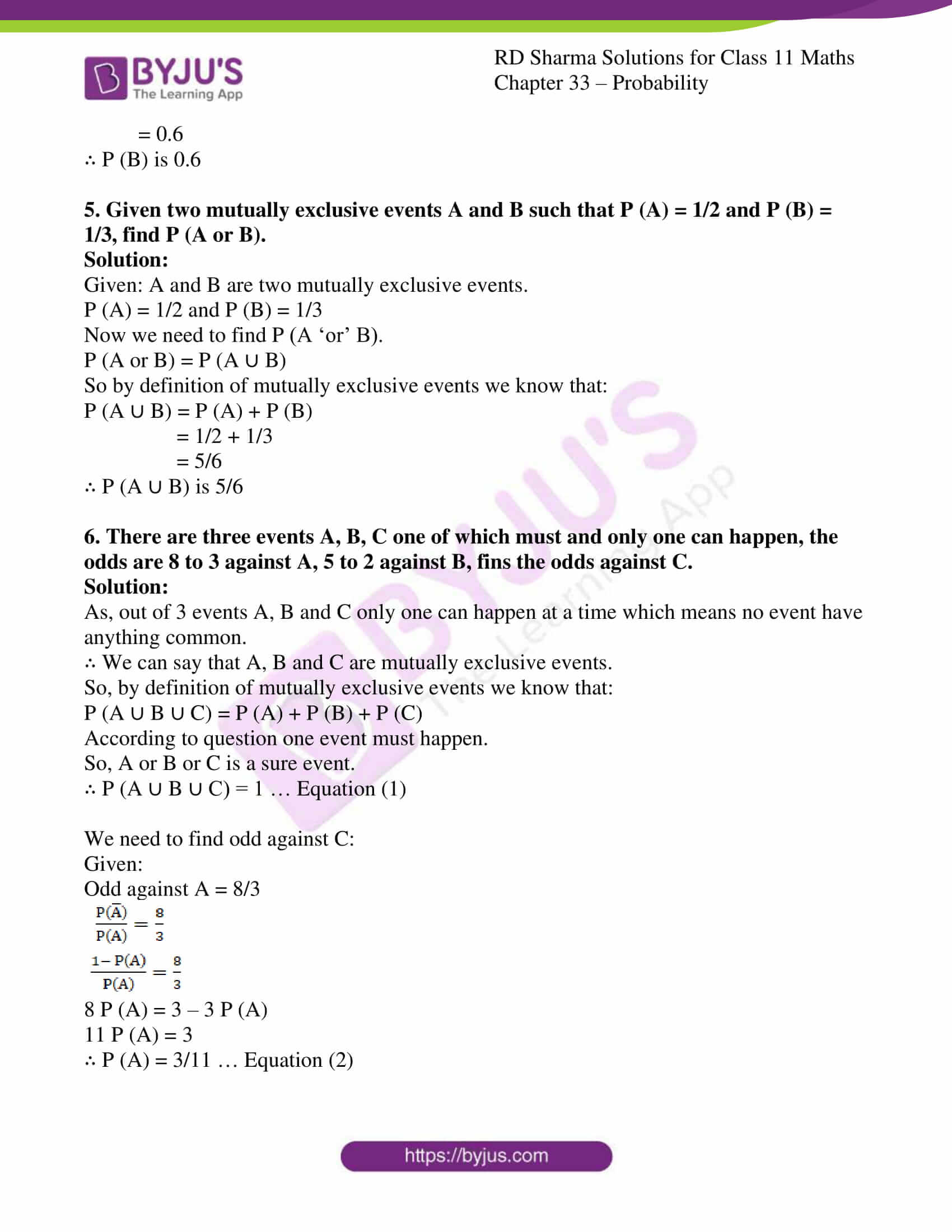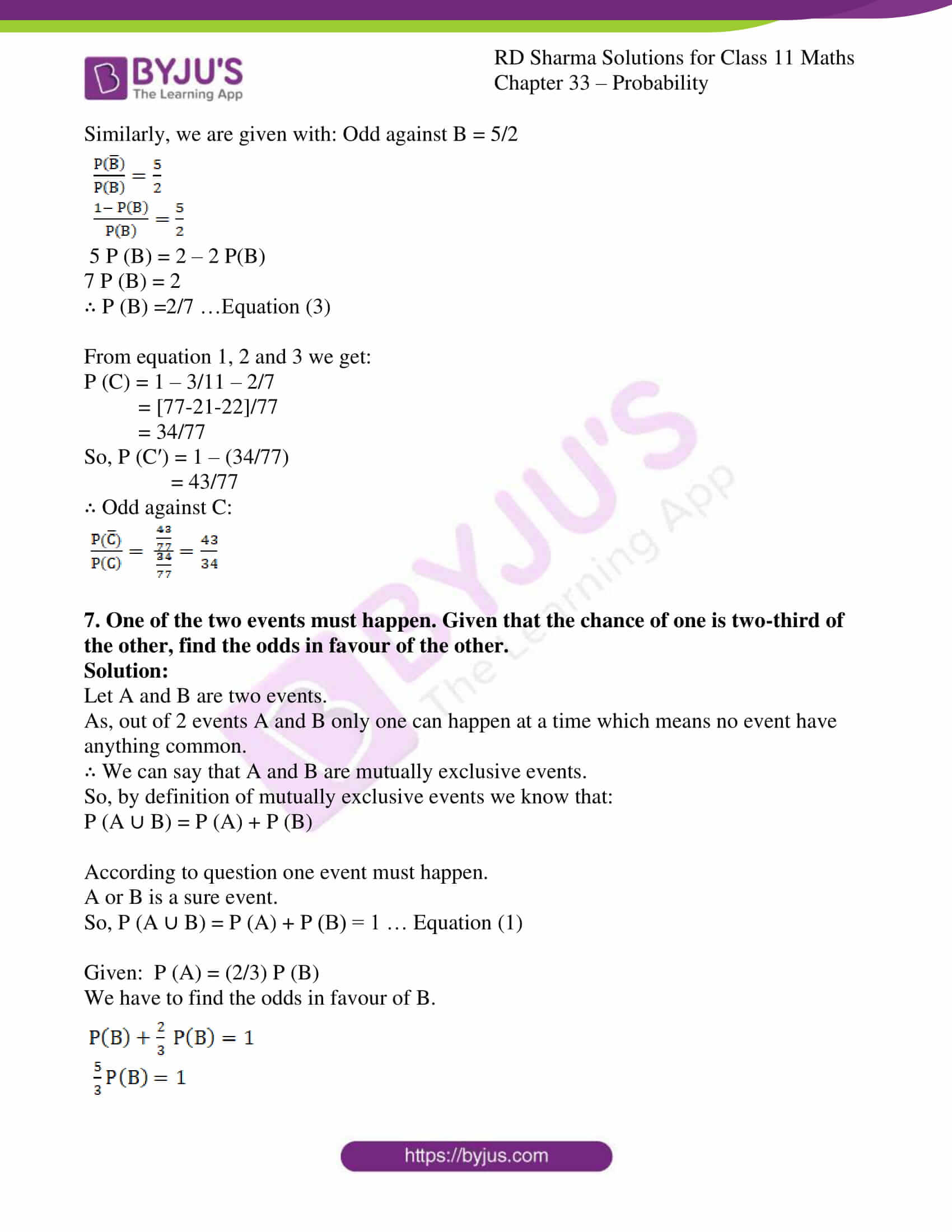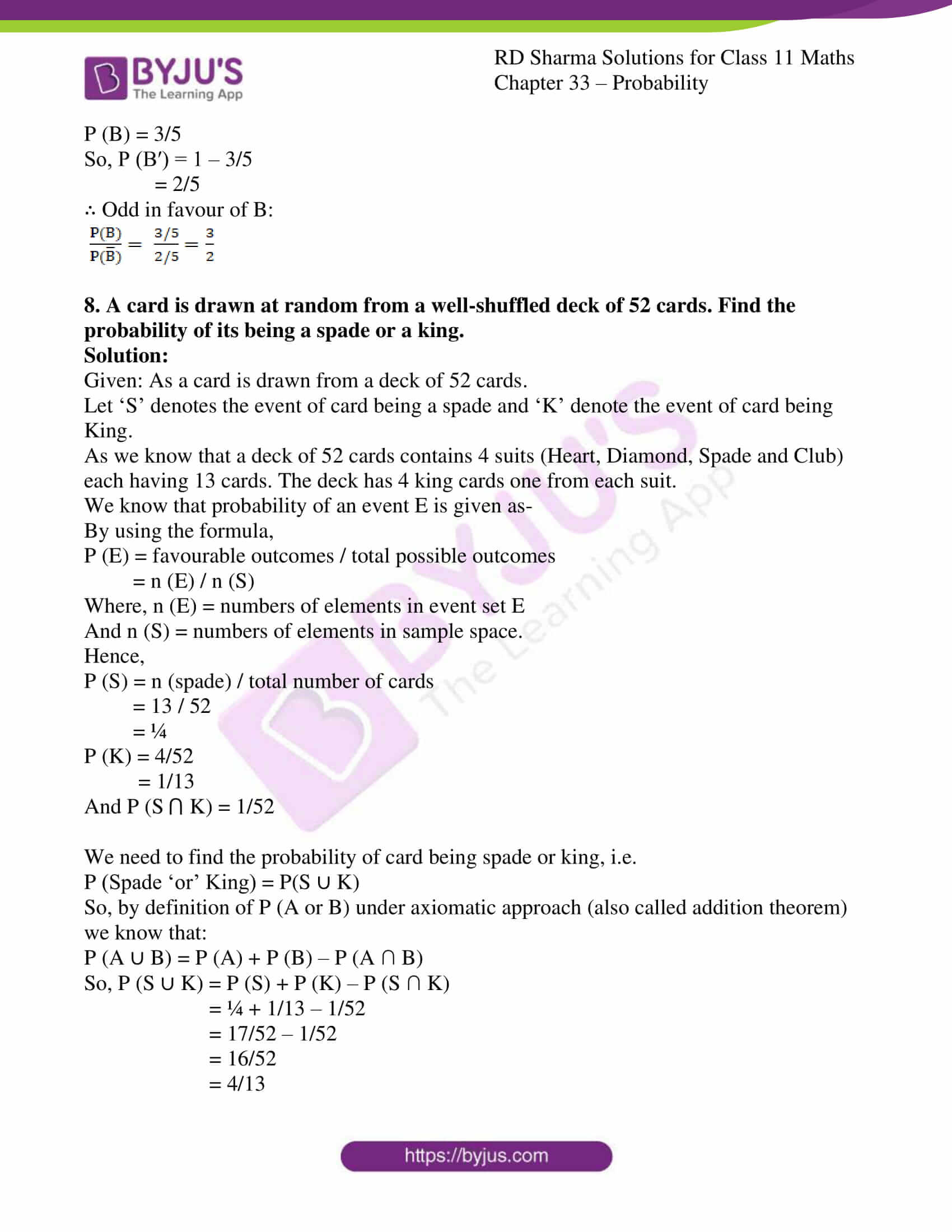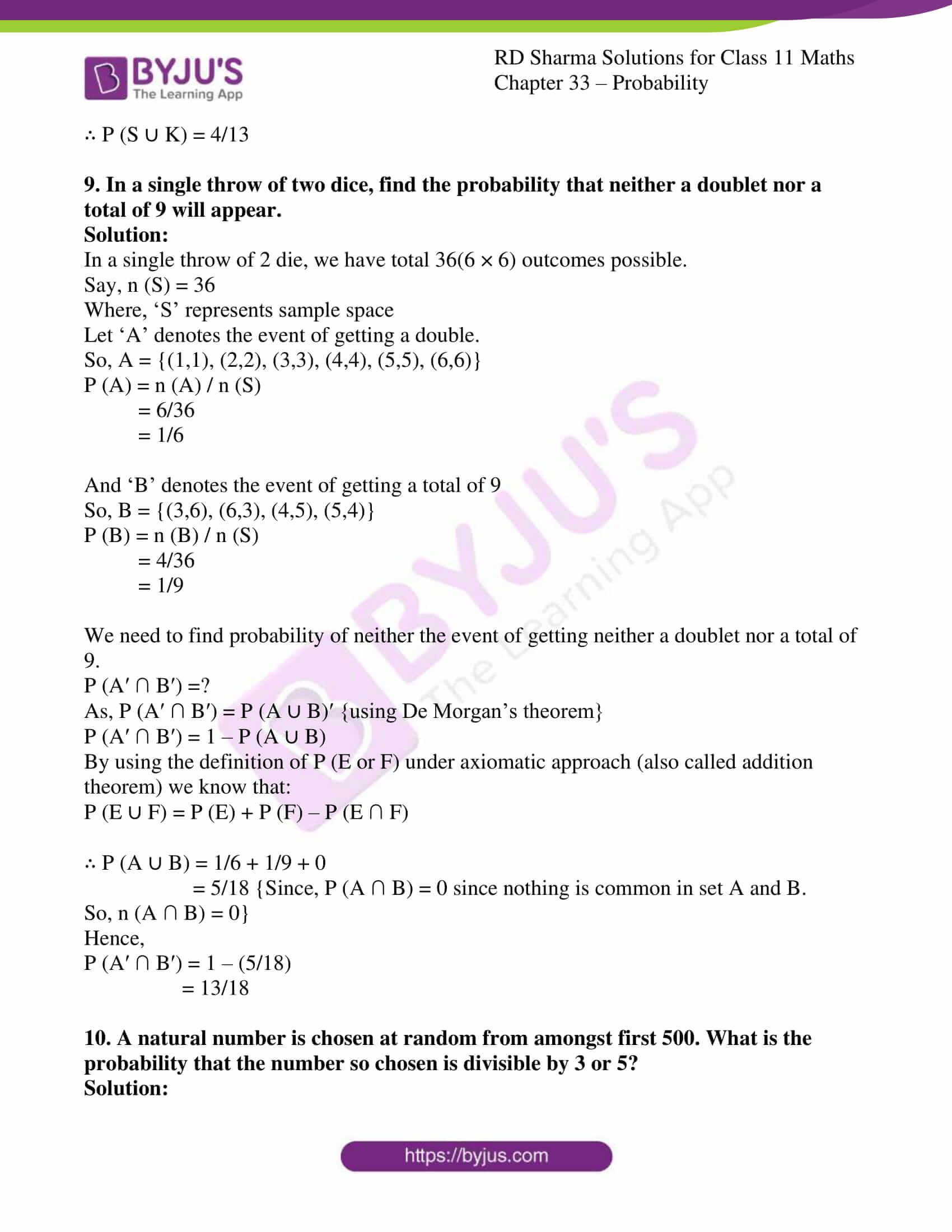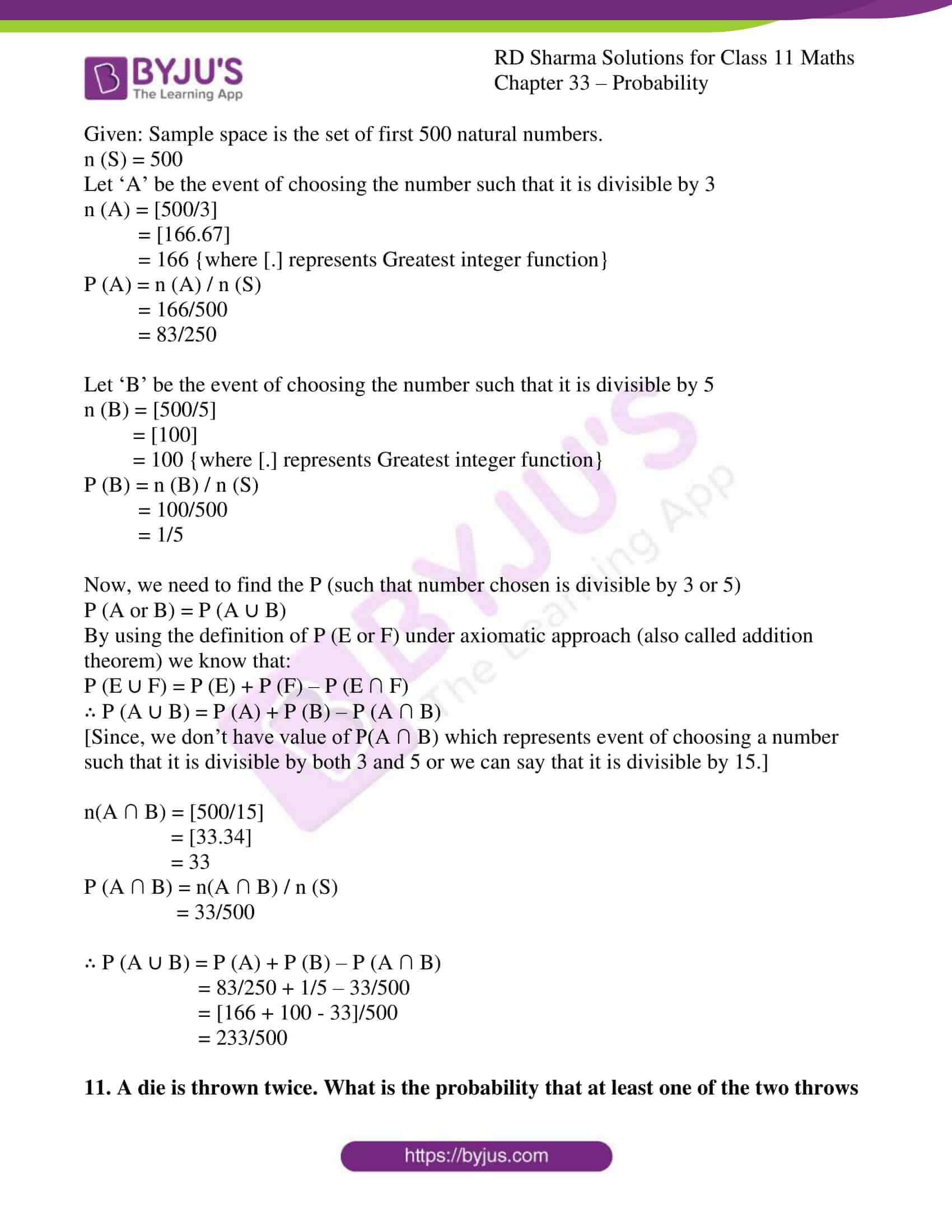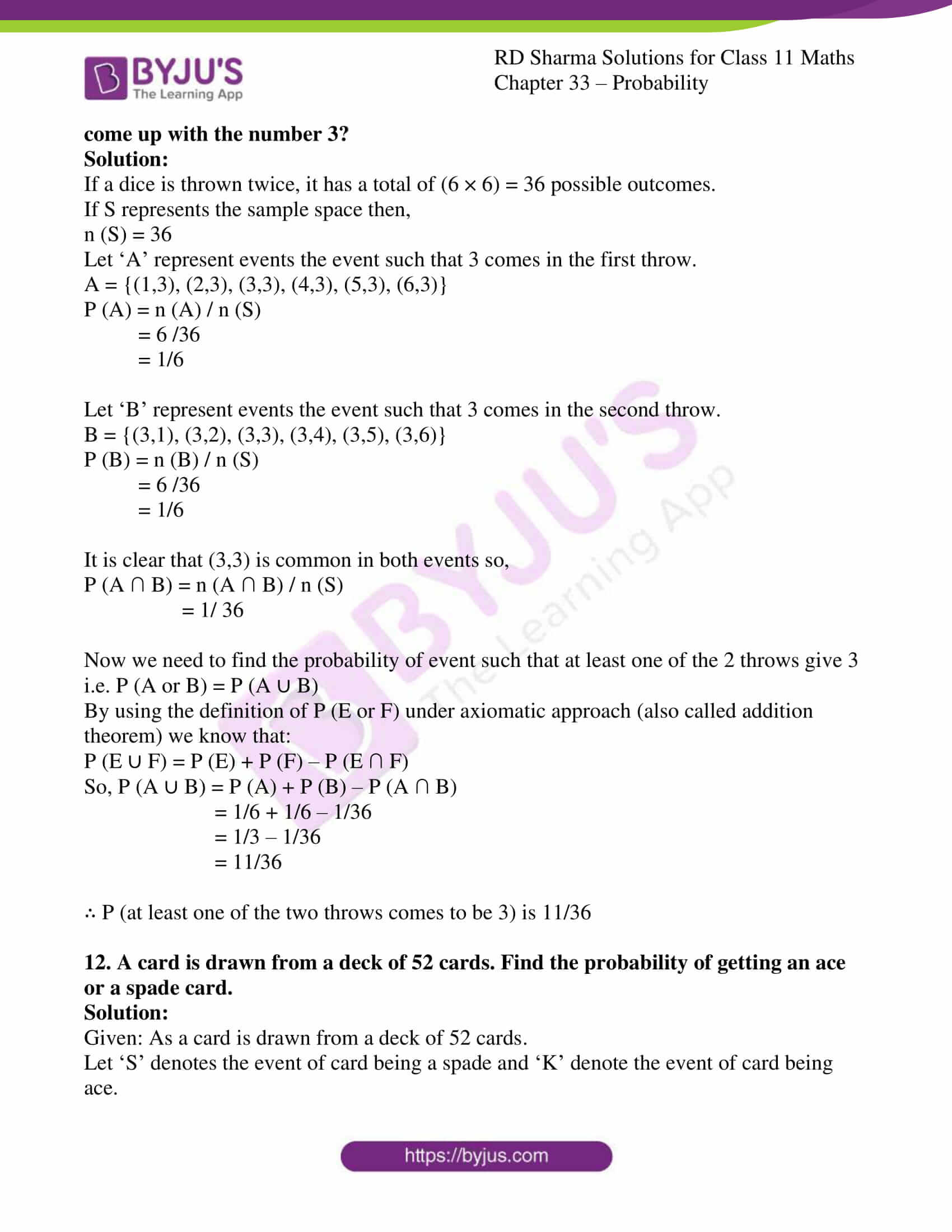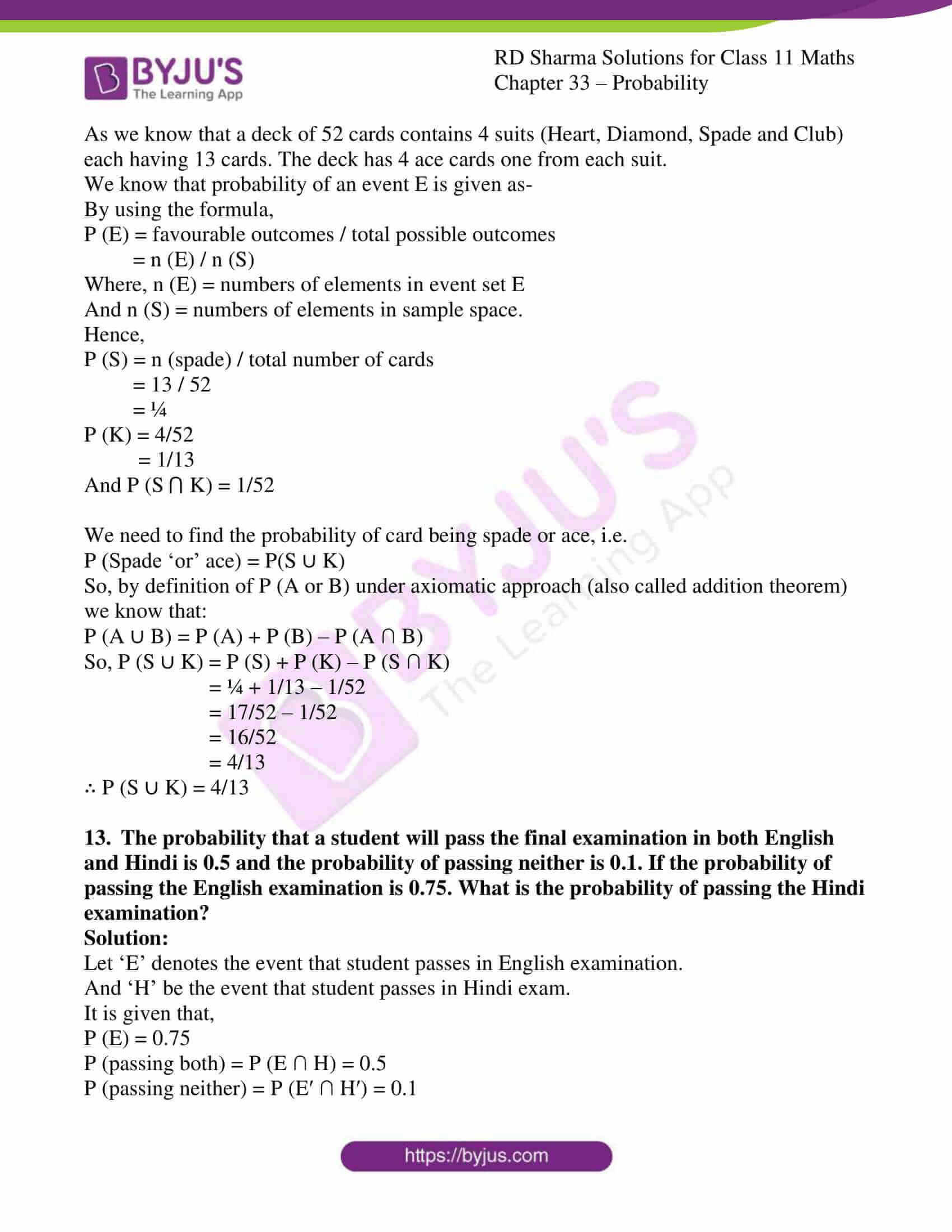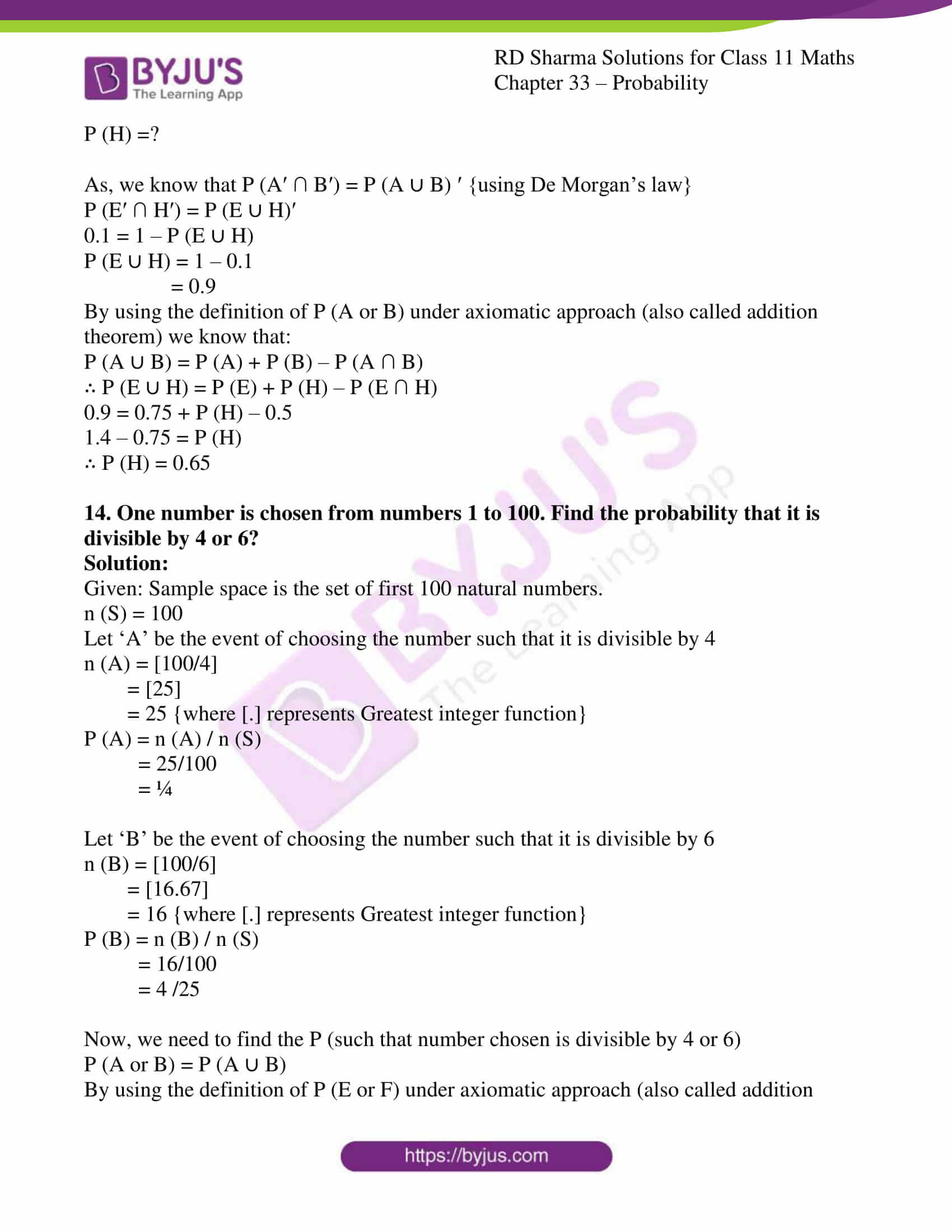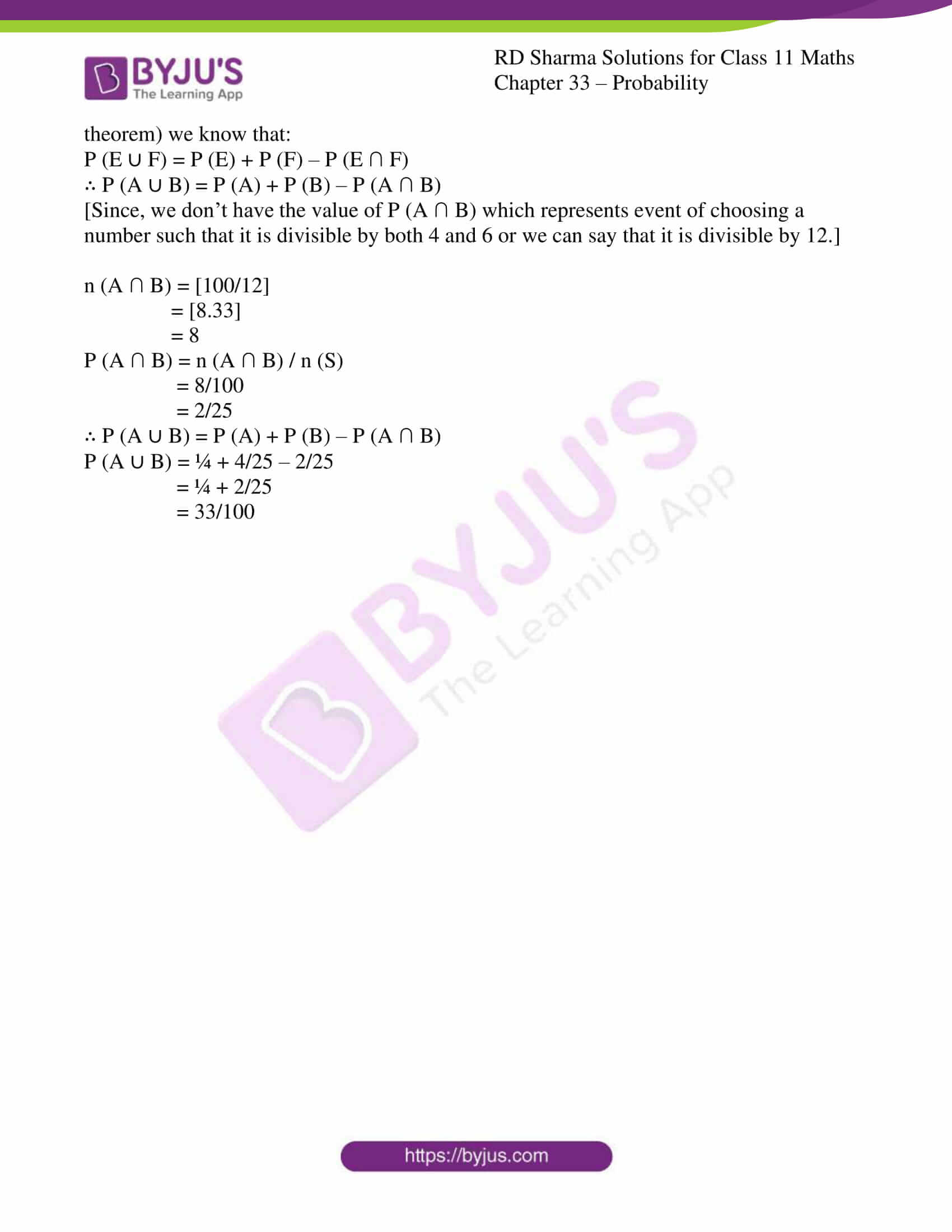### Access answers to RD Sharma Solutions for Class 11 Maths Chapter 33 – Probability

#### EXERCISE 33.1 PAGE NO: 33.6

1. A coin is tossed once. Write its sample space.

Solution:

Given: A coin is tossed once.

We know that, the coin is tossed only once.

Then, there are two probabilities either Head (H) or Tail (T).

So,

S = {H, T}

∴ The sample space is {H, T}

2. If a coin is tossed two times, describe the sample space associated to this experiment.

Solution:

Given: If Coin is tossed twice times.

We know that, two coins are tossed, that means two probabilities will occur at same time.

So,

S = {HT, TH, HH, TT}

∴ Sample space is {HT, HH, TT, TH}

3. If a coin is tossed three times (or three coins are tossed together), then describe the sample space for this experiment.

Solution:

Given: If a coin is tossed three times.

We know that, the coins are tossed three times, and then the no. of samples is

23 = 8

So,

S = {HHH, TTT, HHT, HTH, THH, HTT, THT, TTH}

∴ The sample space is {HHH, TTT, HHT, HTH, THH, HTT, THT, TTH}

4. Write the sample space for the experiment of tossing a coin four times.

Solution:

Given: A coin is tossed four times.

We know that, the coins is tossed four time, then the no. of samples

24 = 16

So,

S = {HHHH, TTTT, HHHT, HHTH, HTHH, THHH, HHTT, HTTH, HTHT, THHT, THTH, TTHH, HTTT, THTT, TTHT, TTTH}

∴ The sample space is {HHHH, TTTT, HHHT, HHTH, HTHH, THHH, HHTT, HTTH, HTHT, THHT, THTH, TTHH, HTTT, THTT, TTHT, TTTH}

5. Two dice are thrown. Describe the sample space of this experiment.

Solution:

Given: Two dice are thrown.

We know there are 6 faces on a dice. Which contains (1, 2, 3, 4, 5, 6).

Here two dice are thrown, and then we have two faces of dice (one of each).

So, the total sample space will be 62 = 36

∴ The sample space is:

S = {(1, 1), (1, 2), (1, 3), (1, 4), (1, 5), (1, 6), (2, 1), (2, 2), (2, 3), (2, 4), (2, 5), (2, 6), (3, 1), (3, 2), (3, 3), (3, 4), (3, 5), (3, 6), (4, 1), (4, 2), (4, 3), (4, 4), (4, 5), (4, 6), (5, 1), (5, 2), (5, 3), (5, 4), (5, 6), (5, 5), (6, 1), (6, 2), (6, 3), (6, 4), (6, 5), (6, 6)}

6. What is the total number of elementary events associated to the random experiment of throwing three dice together?

Solution:

Given: Three dice is rolled together.

We know that, three dice are thrown together. And, there are 6 faces on die,

So, the total numbers of elementary event on throwing three dice are

6 × 6 × 6 = 216

∴ The total number of elementary events are 216

7. A coin is tossed and then a die is thrown. Describe the sample space for this experiment.

Solution:

Given: A coin is tossed and a die is thrown.

We know that, the coin is tossed and die is thrown.

So, when coin is tossed there will be 2 events either Head or Tail.

And, when die is thrown then there will be 6 faces (1, 2, 3, 4, 5, 6)

Then,

The total number of Sample space together is 2 × 6 = 12

S = {(H, 1), (H, 2), (H, 3), (H, 4), (H, 5), (H, 6), (T, 1), (T, 2), (T, 3), (T, 4), (T, 5), (T, 6)}

∴ The sample space are {(H, 1), (H, 2), (H, 3), (H, 4), (H, 5), (H, 6), (T, 1), (T, 2), (T, 3), (T, 4), (T, 5), (T, 6)}

8. A coin is tossed and then a die is rolled only in case a head is shown on the coin. Describe the sample space for this experiment.

Solution:

Given: A coin is tossed and the die is rolled.

We know that, we have a coin and a die,

So, when coin is tossed there will be 2 events Head and tail,

According to question, If Head occurs on coin then Die will be rolled out otherwise not.

So, the sample spaces are:

S = {(T, (H, 1), (H, 2), (H, 3), (H, 4), (H, 5), (H, 6)}

∴ The sample space is {T, (H, 1), (H, 2), (H, 3), (H, 4), (H, 5), (H, 6)1}

9. A coin is tossed twice. If the second throw results in a tail, a die is thrown. Describe the sample space for this experiment.

Solution:

Given: A coin is tossed twice. If the second throw results in a tail, a die is thrown.

When a coin tossed twice, then sample spaces for only coin will be: {HH, TT, HT, TH}

Now, according to question, when we get Tail in second throw, then a dice is thrown.

So, the total number of elementary events are 2 + (2×6) = 14

And sample space will be

S = {HH, TH, (HT, 1), (HT, 2), (HT, 3), (HT, 4), (HT, 5), (HT, 6), (TT, 1), (TT, 2), (TT, 3), (TT, 4), (TT, 5), (TT, 6)}

∴ The sample space is {HH, TH, (HT, 1), (HT, 2), (HT, 3), (HT, 4), (HT, 5), (HT, 6), (TT, 1), (TT, 2), (TT, 3), (TT, 4), (TT, 5), (TT, 6)}

10. An experiment consists of tossing a coin and then tossing it second time if head occurs. If a tail occurs on the first toss, then a die is tossed once. Find the sample space.

Solution:

Given: A coin is tossed and a die is rolled.

In the given experiment, coin is tossed and if the outcome is tail then, die will be rolled.

The possible outcome for coin is 2 = {H, T}

And, the possible outcome for die is 6 = {1, 2, 3, 4, 5, 6}

If the outcome for the coin is tail then sample space is S1= {(T, 1) (T, 2) (T, 3) (T, 4) (T, 5) (T, 6)}

If the outcome is head then the sample space is S2 = {(H, H) (H, T)}

So, the required outcome sample space is S = S1 ⋃ S2

S = {(T, 1) (T, 2) (T, 3) (T, 4) (T, 5) (T, 6) (H, H) (H, T)}

∴ The sample space for the given experiment is {(T, 1) (T, 2) (T, 3) (T, 4) (T, 5) (T, 6) (H, H) (H, T)}

11. A coin is tossed. If it shows tail, we draw a ball from a box which contains 2 red 3 black balls; it shows head, we throw a die. Find the sample space of this experiment.

Solution:

Given: A coin is tossed and there is box which contains 2 red and 3 black balls.

When coin is tossed, there are 2 outcomes {H, T}

According to question, if tail turned up, the ball is drawn from a box.

So, sample for this experiment S1 = {(T, R1) (T, R2) (T, B1) (T, B2) (T, B3)}

Now, If Head is turned up, and then die is rolled.

So, sample space for this experiment S2 = {(H, 1) (H, 2) (H, 3) (H, 4) (H, 5) (H, 6)}

The required sample space will be S = S1 ⋃ S2

So,

S = {(T, R1), (T, R2), (T, B1), (T, B2), (T, B3), (H, 1), (H, 2), (H, 3), (H, 4), (H, 5), (H, 6)}

∴ S is the elementary events associated with the given experiment.

12. A coin is tossed repeatedly until a tail comes up for the first time. Write the sample space for this experiment.

Solution:

Given: A coin is tossed repeatedly until comes up for the first time.

In the given Experiment, a coin is tossed and if the outcome is tail the experiment is over.

And, if the outcome is Head then the coin is tossed again.

In the second toss also if the outcome is tail then experiment is over, otherwise coin is tossed again.

This process continues indefinitely

So, the sample space for this experiment is

S = {T, HT, HHT, HHHT, HHHHT…}

∴ The sample space for the given experiment is {T, HT, HHT, HHHT, HHHHT…}

#### EXERCISE 33.2 PAGE NO: 33.15

1. A coin is tossed. Find the total number of elementary events and also the total number of events associated with the random experiment.

Solution:

Given: A coin is tossed.

When a coin is tossed, there will be two possible outcomes, Head (H) and Tail (T).

Since, the no. of elementary events is 2 {H, T}

We know, if there are n elements in a set, then the number of total element in its subset is 2n.

So, the total number of the experiment is 4,

There are 4 subset of S = {H}, {T}, {H, T} and Փ

∴ There are total 4 events in a given experiment.

2. List all events associated with the random experiment of tossing of two coins. How many of them are elementary events?

Solution:

Given: Two coins are tossed once.

We know, when two coins are tossed then the no. of possible outcomes are 22 = 4

So, the Sample spaces are {HH, HT, TT, TH}

∴ There are total 4 events associated with the given experiment.

3. Three coins are tossed once. Describe the following events associated with this random experiment:
A = Getting three heads, B = Getting two heads and one tail, C = Getting three tails, D = Getting a head on the first coin.
(i) Which pairs of events are mutually exclusive?
(ii) Which events are elementary events?
(iii) Which events are compound events?

Solution:

Given: There are three coins tossed once.

When three coins are tossed, then the sample spaces are:

S = {HHH, HHT, HTH, HTT, THH, THT, TTH, TTT}

So, according to the question,

A = {HHH}

B = {HHT, HTH, THH}

C = {TTT}

D = {HHH, HHT, HTH, HTT}

Now, A⋂ B = Փ,

A ⋂ C = Փ,

A ⋂ D = {HHH}

B⋂ C = Փ,

B ⋂ D = {HHT, HTH}

C ⋂ D = Փ

We know that, if the intersection of two sets are null or empty it means both the sets are Mutually Exclusive.

(i) Events A and B, Events A and C, Events B and C and events C and D are mutually exclusive.

(ii) Here, We know, if an event has only one sample point of a sample space, then it is called elementary events.

So, A and C are elementary events.

(iii) If there is an event that has more than one sample point of a sample space, it is called a compound event.

Since, B ⋂ D = {HHT, HTH}

So, B and D are compound events.

4. In a single throw of a die describe the following events:
(i) A = Getting a number less than 7
(ii) B = Getting a number greater than 7
(iii) C = Getting a multiple of 3
(iv) D = Getting a number less than 4
(v) E = Getting an even number greater than 4.
(vi) F = Getting a number not less than 3.
Also, find A ∪ B, A ∩ B, B ∩ C, E ∩ F, D ∩ F and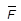.

Solution:

Given: A dice is thrown once.

Let us find the given events, and also find A ∪ B, A ∩ B, B ∩ C, E ∩ F, D ∩ F and.

S = {1, 2, 3, 4, 5, 6}

According to the subparts of the question, we have certain events as:

(i) A = getting a number below 7

So, the sample spaces for A are:

A = {1, 2, 3, 4, 5, 6}

(ii) B = Getting a number greater than 7

So, the sample spaces for B are:

B = {Փ}

(iii) C = Getting multiple of 3

So, the Sample space of C is

C = {3, 6}

(iv) D = Getting a number less than 4

So, the sample space for D is

D = {1, 2, 3}

(v) E = Getting an even number greater than 4.

So, the sample space for E is

E = {6}

(vi) F = Getting a number not less than 3.

So, the sample space for F is

F = {3, 4, 5, 6}

Now,

A = {1, 2, 3, 4, 5, 6} and B = {Փ}

A ⋃ B = {1, 2, 3, 4, 5, 6}

A = {1, 2, 3, 4, 5, 6} and B = {Փ}

A ⋂ B = {Փ}

B = {Փ} and C = {3, 6}

B ⋂ C = {Փ}

F = {3, 4, 5, 6} and E = {6}

E ⋂ F = {6}

E = {6} and D = {1, 2, 3}

D ⋂ F = {3}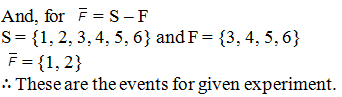5. Three coins are tossed. Describe
(i) two events A and B which are mutually exclusive.
(ii) three events A, B and C which are mutually exclusive and exhaustive.
(iii) two events A and B which are not mutually exclusive.
(iv) two events A and B which are mutually exclusive but not exhaustive.

Solution:

Given: Three coins are tossed.

When three coins are tossed, then the sample space is

S = {HHH, HHT, HTH, HTT, THH, THT, TTH, TTT}

Now, the subparts are:

(i) The two events which are mutually exclusive are when,

A: getting no tails

Then, A = {HHH} and B = {TTT}

So, the intersection of this set will be null. Or, the sets are disjoint.

(ii) Three events which are mutually exclusive and exhaustive are:

C: getting at least two head

So, A = {TTT} B = {TTH, THT, HTT} and C = {HHH, HHT, HTH, THH}

Since, A ⋃ B = B ⋂ C = C ⋂ A = Փ and

A⋃ B⋃ C = S

(iii) The two events that are not mutually exclusive are:

B: getting at least 2 heads

So, A = {HHH} B = {HHH, HHT, HTH, THH}

Hence, A ⋂ B = {HHH} = Փ

(iv) The two events which are mutually exclusive but not exhaustive are:

B: getting exactly one tail

So, A = {HTT, THT, TTH} and B = {HHT, HTH, THH}

It is because A ⋂ B = Փ but A⋃ B ≠ S

6. A die is thrown twice. Each time the number appearing on it is recorded. Describe the following events:
(i) A = Both numbers are odd.
(ii) B = Both numbers are even
(iii) C = sum of the numbers is less than 6.
Also, find A ∪ B, A ∩ B, A ∪ C, A ∩ C. Which pairs of events are mutually exclusive?

Solution:

Given: A dice is thrown twice. And each time number appearing on it is recorded.

When the dice is thrown twice then the number of sample spaces are 62 = 36

Now,

The possibility both odd numbers are:

A = {(1, 1), (1, 3), (1, 5), (3, 1), (3, 3), (3, 5), (5, 1), (5, 3), (5, 5)}

Since, possibility of both even numbers is:

B = {(2, 2), (2, 4), (2, 6), (4, 2), (4, 4), (4, 6), (6, 2), (6, 4), (6, 6)}

And, possible outcome of sum of the numbers is less than 6.

C = {(1, 1)(1, 2)(1, 3)(1, 4)(2, 1)(2, 2)(2, 3)(3, 1)(3, 2)(4, 1)}

Hence,

(AՍB) = {(1, 1), (1, 3), (1, 5), (3, 1), (3, 3), (3, 5), (5, 1), (5, 3), (5, 5) (2, 2)(2, 4)(2, 6)(4, 2)(4, 4)(4, 6)(6, 2)(6, 4)(6, 6)}

(AՌB) = {Փ}

(AUC) = {(1, 1), (1, 3), (1, 5), (3, 1), (3, 3), (3, 5), (5, 1), (5, 3), (5, 5) (1, 2)(1, 4)(2, 1)(2, 2)(2, 3)(3, 1)(3, 2)(4, 1)}

(AՌC) = {(1, 1), (1, 3), (3, 1)}

∴ (AՌB) = Փ and (AՌC) ≠ Փ, A and B are mutually exclusive, but A and C are not.

#### EXERCISE 33.3 PAGE NO: 33.45

1. Which of the following cannot be valid assignment of probability for elementary events or outcomes of sample space S = {w1, w2, w3, w4, w5, w6, w7}: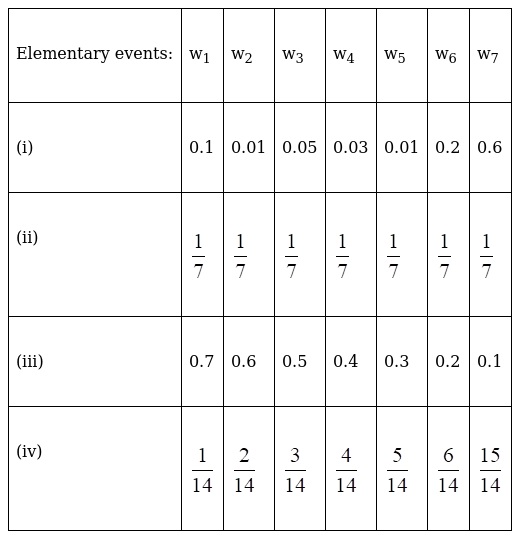Solution:

For each event to be a valid assignment of probability.

The probability of each event in sample space should be less than 1 and the sum of probability of all the events should be exactly equal to 1.

(i) It is valid as each P (wi) (for i=1 to 7) lies between 0 to 1 and sum of P (w1) =1

(ii) It is valid as each P (wi) (for i=1 to 7) lies between 0 to 1 and sum of P (w1) =1

(iii) It is not valid as sum of P (wi) = 2.8 which is greater than 1

(iv) it is not valid as P (w7) = 15/14 which is greater than 1

2. A die is thrown. Find the probability of getting:
(i) a prime number
(ii) 2 or 4
(iii) a multiple of 2 or 3

Solution:

Given: A die is thrown.

The total number of outcomes is six, n (S) = 6

By using the formula,

P (E) = favourable outcomes / total possible outcomes

(i) Let E be the event of getting a prime number

E = {2, 3, 5}

n (E) = 3

P (E) = n (E) / n (S)

= 3 / 6

= ½

(ii) Let E be the event of getting 2 or 4

E = {2, 4}

n (E) = 2

P (E) = n (E) / n (S)

= 2 / 6

= 1/3

(iii) Let E be the event of getting a multiple of 2 or 3

E = {2, 3, 4, 6}

n (E) = 4

P (E) = n (E) / n (S)

= 4 / 6

= 2/3

3. In a simultaneous throw of a pair of dice, find the probability of getting:
(i) 8 as the sum
(ii) a doublet
(iii) a doublet of prime numbers
(iv) an even number on first
(v) a sum greater than 9
(vi) an even number on first
(vii) an even number on one and a multiple of 3 on the other
(viii) neither 9 nor 11 as the sum of the numbers on the faces
(ix) a sum less than 6
(x) a sum less than 7
(xi) a sum more than 7
(xii) neither a doublet nor a total of 10
(xiii) odd number on the first and 6 on the second
(xiv) a number greater than 4 on each die
(xv) a total of 9 or 11
(xvi) a total greater than 8

Solution:

Given: a pair of dice has been thrown, so the number of elementary events in sample space is 62 = 36

n (S) = 36

By using the formula,

P (E) = favourable outcomes / total possible outcomes

(i) Let E be the event that the sum 8 appears

E = {(2, 6) (3, 5) (4, 4) (5, 3) (6, 2)}

n (E) = 5

P (E) = n (E) / n (S)

= 5 / 36

(ii) Let E be the event of getting a doublet

E = {(1, 1) (2, 2) (3, 3) (4, 4) (5, 5) (6, 6)}

n (E) = 6

P (E) = n (E) / n (S)

= 6 / 36

= 1/6

(iii) Let E be the event of getting a doublet of prime numbers

E = {((2, 2) (3, 3) (5, 5)}

n (E) = 3

P (E) = n (E) / n (S)

= 3 / 36

= 1/12

(iv) Let E be the event of getting a doublet of odd numbers

E = {(1, 1) (3, 3) (5, 5)}

n (E) = 3

P (E) = n (E) / n (S)

= 3 / 36

= 1/12

(v) Let E be the event of getting sum greater than 9

E = {(4,6) (5,5) (5,6) (6,4) (6,5) (6,6)}

n (E) = 6

P (E) = n (E) / n (S)

= 6 / 36

= 1/6

(vi) Let E be the event of getting even on first die

E = {(2,1) (2,2) (2,3) (2,4) (2,5) (2,6) (4,1) (4,2) (4,3) (4,4) (4,5) (4,6) (6,1) (6,2) (6,3) (6,4) (6,5) (6,6)}

n (E) = 18

P (E) = n (E) / n (S)

= 18 / 36

= ½

(vii) Let E be the event of getting even on one and multiple of three on other

E = {(2,3) (2,6) (4,3) (4,6) (6,3) (6,6) (3,2) (3,4) (3,6) (6,2) (6,4)}

n (E) = 11

P (E) = n (E) / n (S)

= 11 / 36

(viii) Let E be the event of getting neither 9 or 11 as the sum

E = {(3,6) (4,5) (5,4) (5,6) (6,3) (6,5)}

n (E) = 6

P (E) = n (E) / n (S)

= 6 / 36

= 1/6

(ix) Let E be the event of getting sum less than 6

E = {(1,1) (1,2) (1,3) (1,4) (2,1) (2,2) (2,3) (3,1) (3,2) (4,1)}

n (E) = 10

P (E) = n (E) / n (S)

= 10 / 36

= 5/18

(x) Let E be the event of getting sum less than 7

E = {(1,1) (1,2) (1,3) (1,4) (1,5) (2,1) (2,2) (2,3) (2,4) (3,1) (3,2) (3,3) (4,1) (4,2) (5,1)}

n (E) = 15

P (E) = n (E) / n (S)

= 15 / 36

= 5/12

(xi) Let E be the event of getting more than 7

E = {(2,6) (3,5) (3,6) (4,4) (4,5) (4,6) (5,3) (5,4) (5,5) (5,6) (6,2) (6,3) (6,4) (6,5) (6,6)}

n (E) = 15

P (E) = n (E) / n (S)

= 15 / 36

= 5/12

(xii) Let E be the event of getting neither a doublet nor a total of 10

E′ be the event that either a double or a sum of ten appears

E′ = {(1,1) (2,2) (3,3) (4,6) (5,5) (6,4) (6,6) (4,4)}

n (E′) = 8

P (E′) = n (E′) / n (S)

= 8 / 36

= 2/9

So, P (E) = 1 – P (E′)

= 1 – 2/9

= 7/9

(xiii) Let E be the event of getting odd number on first and 6 on second

E = {(1,6) (5,6) (3,6)}

n (E) = 3

P (E) = n (E) / n (S)

= 3 / 36

= 1/12

(xiv) Let E be the event of getting greater than 4 on each die

E = {(5,5) (5,6) (6,5) (6,6)}

n (E) = 4

P (E) = n (E) / n (S)

= 4 / 36

= 1/9

(xv) Let E be the event of getting total of 9 or 11

E = {(3,6) (4,5) (5,4) (5,6) (6,3) (6,5)}

n (E) = 6

P (E) = n (E) / n (S)

= 6 / 36

= 1/6

(xvi) Let E be the event of getting total greater than 8

E = {(3,6) (4,5) (4,6) (5,4) (5,5) (5,6) (6,3) (6,4) (6,5) (6,6)}

n (E) = 10

P (E) = n (E) / n (S)

= 10 / 36

= 5/18

4. In a single throw of three dice, find the probability of getting a total of 17 or 18

Solution:

Given: The dices are thrown.

By using the formula,

P (E) = favourable outcomes / total possible outcomes

Total number of possible outcomes is 63=216

So, n (S) = 216

Let E be the event of getting total of 17 or 18

E = {(6, 6, 5) (6, 5, 6) (5, 6, 6) (6, 6, 6)}

n (E) = 4

P (E) = n (E) / n (S)

= 4 / 216

= 1/54

5. Three coins are tossed together. Find the probability of getting:
(iii) at least one head and one tail

Solution:

Given: Three coins are tossed together.

By using the formula,

P (E) = favourable outcomes / total possible outcomes

Total number of possible outcomes is 23 = 8

(i) Let E be the event of getting exactly two heads

E = {(H, H, T) (H, T, H) (T, H, H)}

n (E) = 3

P (E) = n (E) / n (S)

= 3 / 8

(ii) Let E be the event of getting at least two heads

E= {(H, H, T) (H, T, H) (T, H, H) (H, H, H)}

n (E)=4

P (E) = n (E) / n (S)

= 4 / 8

= ½

(iii) Let E be the event of getting at least one head and one tail

E = {(H, T, T) (T, H, T) (T, T, H) (H, H, T) (H, T, H) (T, H, H)}

n (E) = 6

P (E) = n (E) / n (S)

= 6 / 8

= ¾

6. What is the probability that an ordinary year has 53 Sundays?

Solution:

Given: A year which includes 52 weeks and one day.

By using the formula,

P (E) = favourable outcomes / total possible outcomes

So, we now have to determine the probability of that one day being Sunday

Total number of possible outcomes is 7

n(S) = 7

E = {M, T, W, T, F, S, SU}

n (E) = 1

P (E) = n (E) / n (S)

= 1 / 7

7. What is the probability that a leap year has 53 Sundays and 53 Mondays?

Solution:

Given: A leap year which includes 52 weeks and two days

By using the formula,

P (E) = favourable outcomes / total possible outcomes

So, we now have to determine the probability of that remaining two days is Sunday and Monday

S = {MT, TW, WT, TF, FS, SSu, SuM}

n (S) = 7

E= {SuM}

n (E) = 1

P (E) = n (E) / n (S)

= 1 / 7

8. A bag contains 8 red and 5 white balls. Three balls are drawn at random. Find the probability that:
(i) All the three balls are white
(ii) All the three balls are red
(iii) One ball is red and two balls are white

Solution:

Given: A bag contains 8 red and 5 white balls.

By using the formula,

P (E) = favourable outcomes / total possible outcomes

Total number of ways of drawing three balls at random is 13C3

n (S) = 286

(i) Let E be the event of getting all white balls

E= {(W) (W) (W)}

n (E)= 5C3=10

P (E) = n (E) / n (S)

= 10 / 286

= 5/143

(ii) Let E be the event of getting all red balls

E = {(R) (R) (R)}

n (E)= 8C3 =56

P (E) = n (E) / n (S)

= 56 / 286

= 28/143

(iii) Let E be the event of getting one red and two white balls

E = {(R…. 80th R)}

n (E)= 8C15C2 = 80

P (E) = n (E) / n (S)

= 80 / 286

= 40/143

9. In a single throw of three dice, find the probability of getting the same number on all the three dice

Solution:

Given: Three dice are rolled over.

By using the formula,

P (E) = favourable outcomes / total possible outcomes

So, we now have to determine the probability of getting the same number on all the three dice

Total number of possible outcomes is 63=216

n (S) = 216

Let E be the event of getting same number on all the three dice

E = {(1,1,1) (2,2,2) (3,3,3) (4,4,4) (5,5,5) (6,6,6)}

n (E) = 6

P (E) = n (E) / n (S)

= 6 / 216

= 1/36

10. Two unbiased dice are thrown. Find the probability that the total of the numbers on the dice is greater than 10

Solution:

Given: Two unbiased dice are thrown.

By using the formula,

P (E) = favourable outcomes / total possible outcomes

So, we now have to determine the probability of getting the sum of digits on dice greater than 10

Total number of possible outcomes is 62=36

n (S) = 36

Let E be the event of getting same number on all the three dice

E = {(5,6) (6,5) (6,6)}

n (E) = 3

P (E) = n (E) / n (S)

= 3 / 36

= 1/12

11. A card is drawn at random from a pack of 52 cards. Find the probability that the card drawn is:

(i) a black king
(ii) either a black card or a king
(iii) black and a king
(iv) a jack, queen or a king
(v) neither an ace nor a king
(vii) neither an ace nor a king
(viii) a diamond card
(ix) not a diamond card
(x) a black card
(xi) not an ace
(xii) not a black card

Solution:

Given: Pack of 52 cards.

By using the formula,

P (E) = favourable outcomes / total possible outcomes

We know that, a card is drawn from a pack of 52 cards, so number of elementary events in the sample space is

n (S) = 52C1 = 52

(i) Let E be the event of drawing a black king

n (E) =2C1 =2 (there are two black kings one of spade and other of club)

P (E) = n (E) / n (S)

= 2 / 52

= 1/26

(ii) Let E be the event of drawing a black card or a king

n (E) = 26C1+4C12C1= 28

[We are subtracting 2 from total because there are two black king which are already counted and to avoid the error of considering it twice.]

P (E) = n (E) / n (S)

= 28 / 52

= 7/13

(iii) Let E be the event of drawing a black card and a king

n (E) =2C1 = 2 (there are two black kings one of spade and other of club)

P (E) = n (E) / n (S)

= 2 / 52

= 1/26

(iv) Let E be the event of drawing a jack, queen or king

n (E) = 4C1+4C1+4C1 = 12

P (E) = n (E) / n (S)

= 12 / 52

= 3/13

(v) Let E be the event of drawing neither a heart nor a king

Now let us consider E′ as the event that either a heart or king appears

n (E′) = 6C1+4C1-1=16 (there is a heart king so it is deducted)

P (E′) = n (E′) / n (S)

= 16 / 52

= 4/13

So, P (E) = 1 – P (E′)

= 1 – 4/13

= 9/13

(vi) Let E be the event of drawing a spade or king

n (E)=13C1+4C1-1=16

P (E) = n (E) / n (S)

= 16 / 52

= 4/13

(vii) Let E be the event of drawing neither an ace nor a king

Now let us consider E′ as the event that either an ace or king appears

n(E′) = 4C1+4C1 = 8

P (E′) = n (E′) / n (S)

= 8 / 52

= 2/13

So, P (E) = 1 – P (E′)

= 1 – 2/13

= 11/13

(viii) Let E be the event of drawing a diamond card

n (E)=13C1=13

P (E) = n (E) / n (S)

= 13 / 52

= ¼

(ix) Let E be the event of drawing not a diamond card

Now let us consider E′ as the event that diamond card appears

n (E′) =13C1=13

P (E′) = n (E′) / n (S)

= 13 / 52

= 1/4

So, P (E) = 1 – P (E′)

= 1 – 1/4

= ¾

(x) Let E be the event of drawing a black card

n (E) =26C1 = 26 (spades and clubs)

P (E) = n (E) / n (S)

= 26 / 52

= ½

(xi) Let E be the event of drawing not an ace

Now let us consider E′ as the event that ace card appears

n (E′) = 4C1 = 4

P (E′) = n (E′) / n (S)

= 4 / 52

= 1/13

So, P (E) = 1 – P (E′)

= 1 – 1/13

=12/13

(xii) Let E be the event of not drawing a black card

n (E) = 26C1 = 26 (red cards of hearts and diamonds)

P (E) = n (E) / n (S)

= 26 / 52

= ½

12. In shutting a pack of 52 playing cards, four are accidently dropped; find the chance that the missing cards should be one from each suit

Solution:

Given: A pack of 52 cards from which 4 are dropped.

By using the formula,

P (E) = favourable outcomes / total possible outcomes

We now have to find the probability that the missing cards should be one from each suit

We know that, from well shuffled pack of cards, 4 cards missed out total possible outcomes are

n (S) = 52C4 = 270725

Let E be the event that four missing cards are from each suite

n (E) = 13C1×13C1×13C1×13C1 = 134

P (E) = n (E) / n (S)

= 134 / 270725

= 2197/20825

13. From a deck of 52 cards, four cards are drawn simultaneously, find the chance that they will be the four honors of the same suit

Solution:

Given: A deck of 52 cards.

By using the formula,

P (E) = favourable outcomes / total possible outcomes

We now have to find the probability that all the face cards of same suits are drawn.

Total possible outcomes are

n (S) = 52C4

Let E be the event that all the cards drawn are face cards of same suit.

n (E)=4×4C4=4

P (E) = n (E) / n (S)

= 4 / 270725

14. Tickets numbered from 1 to 20 are mixed up together and then a ticket is drawn at random. What is the probability that the ticket has a number which is a multiple of 3 or 7?

Solution:

Given: Numbered tickets from 1 to 20.

By using the formula,

P (E) = favourable outcomes / total possible outcomes

To find the probability of the ticket drawn having a number which is a multiple of 3 or 7.

We know that, one ticket is drawn from a lot of mixed number.

Total possible outcomes are

n (S) = 20C1 = 20

Let E be the event of getting ticket which has number that is multiple of 3 or 7

E = {3,6,9,12,15,18,7,14}

n (E) = 8

P (E) = n (E) / n (S)

= 8 / 20

= 2/5

15. A bag contains 6 red, 4 white and 8 blue balls. If three balls are drawn at random, find the probability that one is red, one is white and one is blue

Solution:

Given: A bag containing 6 red, 4 white and 8 blue balls.

By using the formula,

P (E) = favourable outcomes / total possible outcomes

Three balls are drawn so, we have to find the probability that one is red, one is white and one is blue.

Total number of outcomes for drawing 3 balls is 18C3

n (S) = 18C3 = 816

Let E be the event that one red, one white and one blue ball is drawn.

n (E) = 6C14C18C1 = 192

P (E) = n (E) / n (S)

= 192 / 816

= 4/17

16. A bag contains 7 white, 5 black and 4 red balls. If two balls are drawn at random, find the probability that:
(i) both the balls are white
(ii) one ball is black and the other red
(iii) both the balls are of the same colour

Solution:

Given: A bag containing 7 white, 5 black and 4 red balls.

By using the formula,

P (E) = favourable outcomes / total possible outcomes

Two balls are drawn at random, therefore

Total possible outcomes are 16C2

n (S) = 120

(i) Let E be the event of getting both white balls

E = {(W) (W)}

n (E) = 7C2 = 21

P (E) = n (E) / n (S)

= 21 / 120

= 7/40

(ii) Let E be the event of getting one black and one red ball

E = {(B) (R)}

n (E) = 5C14C1 = 20

P (E) = n (E) / n (S)

= 20 / 120

= 1/6

(iii) Let E be the event of getting both balls of same colour

E = {(B) (B)} or {(W) (W)} or {(R) (R)}

n (E) = 7C2+5C2+4C2 = 37

P (E) = n (E) / n (S)

= 37 / 120

17. A bag contains 6 red, 4 white and 8 blue balls. If three balls are drawn at random, find the probability that:
(i) one is red and two are white
(ii) two are blue and one is red
(iii) one is red

Solution:

Given: A bag containing 6 red, 4 white and 8 blue balls.

By using the formula,

P (E) = favourable outcomes / total possible outcomes

Two balls are drawn at random.

Total possible outcomes are 18C3

n (S) = 816

(i) Let E be the event of getting one red and two white balls

E = {(W) (W) (R)}

n (E) = 6C14C2 = 36

P (E) = n (E) / n (S)

= 36 / 816

= 3/68

(ii) Let E be the event of getting two blue and one red

E = {(B) (B) (R)}

n (E) = 8C26C1 = 168

P (E) = n (E) / n (S)

= 168 / 816

= 7/34

(iii) Let E be the event that one of the balls must be red

E = {(R) (B) (B)} or {(R) (W) (W)} or {(R) (B) (W)}

n (E) = 6C14C18C1+6C14C2+6C18C2 = 396

P (E) = n (E) / n (S)

= 396 / 816

= 33/68

18. Five cards are drawn from a pack of 52 cards. What is the chance that these 5 will contain:
(i) just one ace
(ii) at least one ace?

Solution:

Given: Five cards are drawn from a pack of 52 cards.

By using the formula,

P (E) = favourable outcomes / total possible outcomes

Five cards are drawn at random,

Total possible outcomes are 52C5

n (S) = 2598960

(i) Let E be the event that exactly only one ace is present

n (E) = 4C148C4 = 778320

P (E) = n (E) / n (S)

= 778320 / 2598960

= 3243/10829

(ii) Let E be the event that at least one ace is present

E = {1 or 2 or 3 or 4 ace(s)}

n (E) = 4C148C4+4C248C3+4C348C2+4C448C1 = 886656

P (E) = n (E) / n (S)

= 886656 / 2598960

= 18472/54145

19. The face cards are removed from a full pack. Out of the remaining 40 cards, 4 are drawn at random. What is the probability that they belong to different suits?

Solution:

Given: The face cards are removed from a full pack of 52.

By using the formula,

P (E) = favourable outcomes / total possible outcomes

Four cards are drawn from the remaining 40 cards, so we have to find the probability that all of them belong to different suit.

Total possible outcomes of drawing four cards are 40C4

n (S) =  40C4 = 91390

Let E be the event that 4 cards belong to different suit.

n (E) = 10C110C110C110C1 = 10000

P (E) = n (E) / n (S)

= 10000 / 91390

= 1000/9139

20. There are four men and six women on the city councils. If one council member is selected for a committee at random, how likely is that it is a women?

Solution:

Given: There are four men and six women on the city councils.

By using the formula,

P (E) = favourable outcomes / total possible outcomes

From the city council one person is selected as a council member so, we have to find the probability that it is a woman.

Total possible outcomes of selecting a person is 10C1

n (S)= 10C1 = 10

Let E be the event that it is a woman

n (E) = 6C1 = 6

P (E) = n (E) / n (S)

= 6 / 10

= 3/5

21. A box contains 100bulbs, 20 of which are defective. 10 bulbs are selected for inspection. Find the probability that:
(i) all 10 are defective
(ii) all 10 are good
(iii) at least one is defective
(iv) none is defective

Solution:

Given: A box contains 100bulbs, 20 of which are defective.

By using the formula,

P (E) = favourable outcomes / total possible outcomes

Ten bulbs are drawn at random for inspection,

Total possible outcomes are 100C10

n (S) = 100C10

(i) Let E be the event that all ten bulbs are defective

n (E) = 20C10

P (E) = n (E) / n (S)

= 20C10 / 100C10

(ii) Let E be the event that all ten good bulbs are selected

n (E) = 80C10

P (E) = n (E) / n (S)

= 80C10 / 100C10

(iii) Let E be the event that at least one bulb is defective

E= {1,2,3,4,5,6,7,8,9,10} where 1,2,3,4,5,6,7,8,9,10 are the number of defective bulbs

Let E′ be the event that none of the bulb is defective

n (E′) = 80C10

P (E′) = n (E′) / n (S)

= 80C10 / 100C10

So, P (E) = 1 – P (E′)

= 1 – 80C10 / 100C10

(iv) Let E be the event that none of the selected bulb is defective

n (E) = 80C10

P (E) = n (E) / n (S)

= 80C10 / 100C10

22. Find the probability that in a random arrangement of the letters of the word ‘SOCIAL’ vowels come together

Solution:

Given: The word ‘SOCIAL’.

By using the formula,

P (E) = favourable outcomes / total possible outcomes

In the random arrangement of the alphabets of word “SOCIAL” we have to find the probability that vowels come together.

Total possible outcomes of arranging the alphabets are 6!

n (S) = 6!

Let E be the event that vowels come together

Number of vowels in SOCIAL is A, I, O

So, number of ways to arrange them where, (A, I, O) come together

n (E) = 4! × 3!

P (E) = n (E) / n (S)

= [4! × 3!] / 6!

= 1/5

23. The letters of the word ‘CLIFTON’ are placed at random in a row. What is the chance that two vowels come together?

Solution:

Given: The word ‘CLIFTON’.

By using the formula,

P (E) = favourable outcomes / total possible outcomes

In the random arrangement of the alphabets of word “CLIFTON” we have to find the probability that vowels come together.

Total possible outcomes of arranging the alphabets are 7!

n (S) =7!

Let E be the event that vowels come together

Number of vowels in CLIFTON is I, O

Number of ways to arrange them where, (I, O) come together

n (E)= 6! × 2!

P (E) = n (E) / n (S)

= [6! × 2!] / 7!

= 2/7

#### EXERCISE 33.4 PAGE NO: 33.67

1. (a) If A and B be mutually exclusive events associated with a random experiment such that P (A) = 0.4 and P (B) = 0.5, then find:
(i) P(A ∪ B)Solution:

Given: A and B are two mutually exclusive events.

P (A) = 0.4 and P (B) = 0.5

By definition of mutually exclusive events we know that:

P (A ∪ B) = P (A) + P (B)

Now, we have to find

(i) P (A ∪ B) = P (A) + P (B) = 0.5 + 0.4 = 0.9

(ii) P (A′ ∩ B′) = P (A ∪ B)′ {using De Morgan’s Law}

P (A′ ∩ B′) = 1 – P (A ∪ B)

= 1 – 0.9

= 0.1

(iii) P (A′ ∩ B) [This indicates only the part which is common with B and not A.

Hence this indicates only B]

P (only B) = P (B) – P (A ∩ B)

As A and B are mutually exclusive so they don’t have any common parts.

P (A ∩ B) = 0

∴ P (A′ ∩ B) = P (B) = 0.5

(iv) P (A ∩ B′) [This indicates only the part which is common with A and not B.

Hence this indicates only A]

P (only A) = P (A) – P (A ∩ B)

As A and B are mutually exclusive so they don’t have any common parts.

P (A ∩ B) = 0

∴ P (A ∩ B′) = P (A) = 0.4

(b) A and B are two events such that P (A) = 0.54, P (B) = 0.69 and P (A ∩ B) = 0.35. Find (i) P (A ∪ B)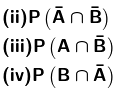Solution:

Given: A and B are two events.

P (A) = 0.54, P (B) = 0.69 and P (A ∩ B) = 0.35

By definition of P (A or B) under axiomatic approach we know that:

P (A ∪ B) = P (A) + P (B) – P (A ∩ B)

Now we have to find:

(i) P (A ∪ B) = P (A) + P (B) – P (A ∩ B)

= 0.54 + 0.69 – 0.35

= 0.88

(ii) P (A′ ∩ B′) = P (A ∪ B)′ {using De Morgan’s Law}

P (A′ ∩ B′) = 1 – P (A ∪ B)

= 1 – 0.88

= 0.12

(iii) P (A ∩ B′) [This indicates only the part which is common with A and not B.

Hence this indicates only A]

P (only A) = P (A) – P (A ∩ B)

∴ P (A ∩ B′) = P (A) – P (A ∩ B)

= 0.54 – 0.35

= 0.19

(iv) P (A′ ∩ B) [This indicates only the part which is common with B and not A.

Hence this indicates only B]

P (only B) = P (B) – P (A ∩ B)

∴ P (A′ ∩ B) = P (B) – P (A ∩ B)

= 0.69 – 0.35

= 0.34

(c) Fill in the blanks in the following table: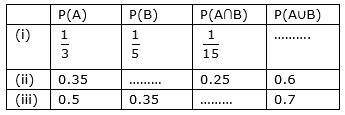Solution:

(i) By definition of P (A or B) under axiomatic approach we know that:

P (A ∪ B) = P (A) + P (B) – P (A ∩ B)

By using data from table, we get:

∴ P (A ∪ B) = 1/3 + 1/5 – 1/15

= 8/15 – 1/15

= 7/15

(ii) By definition of P (A or B) under axiomatic approach we know that:

P (A ∪ B) = P (A) + P (B) – P (A ∩ B)

P (B) = P (A ∪ B) + P (A ∩ B) – P (A)

By using data from table, we get:

∴ P (B) = 0.6 + 0.25 – 0.35

= 0.5

(iii) By definition of P (A or B) under axiomatic approach we know that:

P (A ∪ B) = P (A) + P (B) – P (A ∩ B)

P (A ∩ B) = P (B) + P (A) – P (A ∪ B)

By using data from table, we get:

∴ P (A ∩ B) = 0.5 + 0.35 – 0.7

= 0.15

Hence the table is: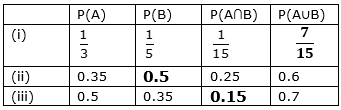2. If A and B are two events associated with a random experiment such that P (A) = 0.3, P (B) = 0.4 and P (A ∪ B) = 0.5, find P (A ∩ B).

Solution:

Given: A and B are two events.

P (A) = 0.3, P (B) = 0.5 and P (A ∪ B) = 0.5

Now we need to find P (A ∩ B).

By definition of P (A or B) under axiomatic approach (also called addition theorem) we know that:

P (A ∪ B) = P (A) + P (B) – P (A ∩ B)

So, P (A ∩ B) = P (A) + P (B) – P (A ∪ B)

P (A ∩ B) = 0.3 + 0.4 – 0.5

= 0.7 – 0.5

= 0.2

∴ P (A ∩ B) is 0.2

3. If A and B are two events associated with a random experiment such that P (A) = 0.5, P (B) = 0.3 and P (A ∩ B) = 0.2, find P (A ∪ B).

Solution:

Given: A and B are two events.

P (A) = 0.5, P (B) = 0.3 and P (A ∩ B) = 0.2

Now we need to find P (A ∪ B).

By definition of P (A or B) under axiomatic approach (also called addition theorem) we know that:

P (A ∪ B) = P (A) + P (B) – P (A ∩ B)

So, P (A ∪ B) = P (A) + P (B) – P (A ∩ B)

P (A ∪ B) = 0.5 + 0.3 – 0.2

= 0.8 – 0.2

= 0.6

∴ P (A ∪ B) is 0.6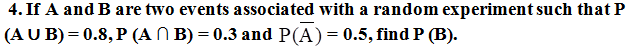Solution:

Given: A and B are two events.

P (A′) = 0.5, P (A ∩ B) = 0.3 and P (A ∪ B) = 0.8

Since, P (A′) = 1 – P (A)

P (A) = 1 – 0.5

= 0.5

Now we need to find P (B).

By definition of P (A or B) under axiomatic approach (also called addition theorem) we know that:

P (A ∪ B) = P (A) + P (B) – P (A ∩ B)

So, P (B) = P (A ∪ B) + P (A ∩ B) – P (A)

P (B) = 0.8 + 0.3 – 0.5

= 1.1 – 0.5

= 0.6

∴ P (B) is 0.6

5. Given two mutually exclusive events A and B such that P (A) = 1/2 and P (B) = 1/3, find P (A or B).

Solution:

Given: A and B are two mutually exclusive events.

P (A) = 1/2 and P (B) = 1/3

Now we need to find P (A ‘or’ B).

P (A or B) = P (A ∪ B)

So by definition of mutually exclusive events we know that:

P (A ∪ B) = P (A) + P (B)

= 1/2 + 1/3

= 5/6

∴ P (A ∪ B) is 5/6

6. There are three events A, B, C one of which must and only one can happen, the odds are 8 to 3 against A, 5 to 2 against B, fins the odds against C.

Solution:

As, out of 3 events A, B and C only one can happen at a time which means no event have anything common.

∴ We can say that A, B and C are mutually exclusive events.

So, by definition of mutually exclusive events we know that:

P (A ∪ B ∪ C) = P (A) + P (B) + P (C)

According to question one event must happen.

So, A or B or C is a sure event.

∴ P (A ∪ B ∪ C) = 1 … Equation (1)

We need to find odd against C:

Given:

Odd against A = 8/3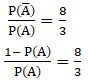8 P (A) = 3 – 3 P (A)

11 P (A) = 3

∴ P (A) = 3/11 … Equation (2)

Similarly, we are given with: Odd against B = 5/2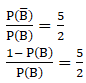5 P (B) = 2 – 2 P(B)

7 P (B) = 2

∴ P (B) =2/7 …Equation (3)

From equation 1, 2 and 3 we get:

P (C) = 1 – 3/11 – 2/7

= [77-21-22]/77

= 34/77

So, P (C′) = 1 – (34/77)

= 43/77

∴ Odd against C: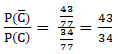7. One of the two events must happen. Given that the chance of one is two-third of the other, find the odds in favour of the other.

Solution:

Let A and B are two events.

As, out of 2 events A and B only one can happen at a time which means no event have anything common.

∴ We can say that A and B are mutually exclusive events.

So, by definition of mutually exclusive events we know that:

P (A ∪ B) = P (A) + P (B)

According to question one event must happen.

A or B is a sure event.

So, P (A ∪ B) = P (A) + P (B) = 1 … Equation (1)

Given: P (A) = (2/3) P (B)

We have to find the odds in favour of B.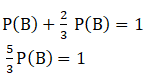P (B) = 3/5

So, P (B′) = 1 – 3/5

= 2/5

∴ Odd in favour of B: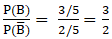8. A card is drawn at random from a well-shuffled deck of 52 cards. Find the probability of its being a spade or a king.

Solution:

Given: As a card is drawn from a deck of 52 cards.

Let ‘S’ denotes the event of card being a spade and ‘K’ denote the event of card being King.

As we know that a deck of 52 cards contains 4 suits (Heart, Diamond, Spade and Club) each having 13 cards. The deck has 4 king cards one from each suit.

We know that probability of an event E is given as-

By using the formula,

P (E) = favourable outcomes / total possible outcomes

= n (E) / n (S)

Where, n (E) = numbers of elements in event set E

And n (S) = numbers of elements in sample space.

Hence,

P (S) = n (spade) / total number of cards

= 13 / 52

= ¼

P (K) = 4/52

= 1/13

And P (S ⋂ K) = 1/52

We need to find the probability of card being spade or king, i.e.

P (Spade ‘or’ King) = P(S ∪ K)

So, by definition of P (A or B) under axiomatic approach (also called addition theorem) we know that:

P (A ∪ B) = P (A) + P (B) – P (A ∩ B)

So, P (S ∪ K) = P (S) + P (K) – P (S ∩ K)

= ¼ + 1/13 – 1/52

= 17/52 – 1/52

= 16/52

= 4/13

∴ P (S ∪ K) = 4/13

9. In a single throw of two dice, find the probability that neither a doublet nor a total of 9 will appear.

Solution:

In a single throw of 2 die, we have total 36(6 × 6) outcomes possible.

Say, n (S) = 36

Where, ‘S’ represents sample space

Let ‘A’ denotes the event of getting a double.

So, A = {(1,1), (2,2), (3,3), (4,4), (5,5), (6,6)}

P (A) = n (A) / n (S)

= 6/36

= 1/6

And ‘B’ denotes the event of getting a total of 9

So, B = {(3,6), (6,3), (4,5), (5,4)}

P (B) = n (B) / n (S)

= 4/36

= 1/9

We need to find probability of neither the event of getting neither a doublet nor a total of 9.

P (A′ ∩ B′) =?

As, P (A′ ∩ B′) = P (A ∪ B)′ {using De Morgan’s theorem}

P (A′ ∩ B′) = 1 – P (A ∪ B)

By using the definition of P (E or F) under axiomatic approach (also called addition theorem) we know that:

P (E ∪ F) = P (E) + P (F) – P (E ∩ F)

∴ P (A ∪ B) = 1/6 + 1/9 + 0

= 5/18 {Since, P (A ∩ B) = 0 since nothing is common in set A and B.

So, n (A ∩ B) = 0}

Hence,

P (A′ ∩ B′) = 1 – (5/18)

= 13/18

10. A natural number is chosen at random from amongst first 500. What is the probability that the number so chosen is divisible by 3 or 5?

Solution:

Given: Sample space is the set of first 500 natural numbers.

n (S) = 500

Let ‘A’ be the event of choosing the number such that it is divisible by 3

n (A) = [500/3]

= [166.67]

= 166 {where [.] represents Greatest integer function}

P (A) = n (A) / n (S)

= 166/500

= 83/250

Let ‘B’ be the event of choosing the number such that it is divisible by 5

n (B) = [500/5]

= 

= 100 {where [.] represents Greatest integer function}

P (B) = n (B) / n (S)

= 100/500

= 1/5

Now, we need to find the P (such that number chosen is divisible by 3 or 5)

P (A or B) = P (A ∪ B)

By using the definition of P (E or F) under axiomatic approach (also called addition theorem) we know that:

P (E ∪ F) = P (E) + P (F) – P (E ∩ F)

∴ P (A ∪ B) = P (A) + P (B) – P (A ∩ B)

[Since, we don’t have value of P(A ∩ B) which represents event of choosing a number such that it is divisible by both 3 and 5 or we can say that it is divisible by 15.]

n(A ∩ B) = [500/15]

= [33.34]

= 33

P (A ∩ B) = n(A ∩ B) / n (S)

= 33/500

∴ P (A ∪ B) = P (A) + P (B) – P (A ∩ B)

= 83/250 + 1/5 – 33/500

= [166 + 100 – 33]/500

= 233/500

11. A die is thrown twice. What is the probability that at least one of the two throws come up with the number 3?

Solution:

If a dice is thrown twice, it has a total of (6 × 6) = 36 possible outcomes.

If S represents the sample space then,

n (S) = 36

Let ‘A’ represent events the event such that 3 comes in the first throw.

A = {(1,3), (2,3), (3,3), (4,3), (5,3), (6,3)}

P (A) = n (A) / n (S)

= 6 /36

= 1/6

Let ‘B’ represent events the event such that 3 comes in the second throw.

B = {(3,1), (3,2), (3,3), (3,4), (3,5), (3,6)}

P (B) = n (B) / n (S)

= 6 /36

= 1/6

It is clear that (3,3) is common in both events so,

P (A ∩ B) = n (A ∩ B) / n (S)

= 1/ 36

Now we need to find the probability of event such that at least one of the 2 throws give 3 i.e. P (A or B) = P (A ∪ B)

By using the definition of P (E or F) under axiomatic approach (also called addition theorem) we know that:

P (E ∪ F) = P (E) + P (F) – P (E ∩ F)

So, P (A ∪ B) = P (A) + P (B) – P (A ∩ B)

= 1/6 + 1/6 – 1/36

= 1/3 – 1/36

= 11/36

∴ P (at least one of the two throws comes to be 3) is 11/36

12. A card is drawn from a deck of 52 cards. Find the probability of getting an ace or a spade card.

Solution:

Given: As a card is drawn from a deck of 52 cards.

Let ‘S’ denotes the event of card being a spade and ‘K’ denote the event of card being ace.

As we know that a deck of 52 cards contains 4 suits (Heart, Diamond, Spade and Club) each having 13 cards. The deck has 4 ace cards one from each suit.

We know that probability of an event E is given as-

By using the formula,

P (E) = favourable outcomes / total possible outcomes

= n (E) / n (S)

Where, n (E) = numbers of elements in event set E

And n (S) = numbers of elements in sample space.

Hence,

P (S) = n (spade) / total number of cards

= 13 / 52

= ¼

P (K) = 4/52

= 1/13

And P (S ⋂ K) = 1/52

We need to find the probability of card being spade or ace, i.e.

P (Spade ‘or’ ace) = P(S ∪ K)

So, by definition of P (A or B) under axiomatic approach (also called addition theorem) we know that:

P (A ∪ B) = P (A) + P (B) – P (A ∩ B)

So, P (S ∪ K) = P (S) + P (K) – P (S ∩ K)

= ¼ + 1/13 – 1/52

= 17/52 – 1/52

= 16/52

= 4/13

∴ P (S ∪ K) = 4/13

13. The probability that a student will pass the final examination in both English and Hindi is 0.5 and the probability of passing neither is 0.1. If the probability of passing the English examination is 0.75. What is the probability of passing the Hindi examination?

Solution:

Let ‘E’ denotes the event that student passes in English examination.

And ‘H’ be the event that student passes in Hindi exam.

It is given that,

P (E) = 0.75

P (passing both) = P (E ∩ H) = 0.5

P (passing neither) = P (E′ ∩ H′) = 0.1

P (H) =?

As, we know that P (A′ ∩ B′) = P (A ∪ B) ′ {using De Morgan’s law}

P (E′ ∩ H′) = P (E ∪ H)′

0.1 = 1 – P (E ∪ H)

P (E ∪ H) = 1 – 0.1

= 0.9

By using the definition of P (A or B) under axiomatic approach (also called addition theorem) we know that:

P (A ∪ B) = P (A) + P (B) – P (A ∩ B)

∴ P (E ∪ H) = P (E) + P (H) – P (E ∩ H)

0.9 = 0.75 + P (H) – 0.5

1.4 – 0.75 = P (H)

∴ P (H) = 0.65

14. One number is chosen from numbers 1 to 100. Find the probability that it is divisible by 4 or 6?

Solution:

Given: Sample space is the set of first 100 natural numbers.

n (S) = 100

Let ‘A’ be the event of choosing the number such that it is divisible by 4

n (A) = [100/4]

= 

= 25 {where [.] represents Greatest integer function}

P (A) = n (A) / n (S)

= 25/100

= ¼

Let ‘B’ be the event of choosing the number such that it is divisible by 6

n (B) = [100/6]

= [16.67]

= 16 {where [.] represents Greatest integer function}

P (B) = n (B) / n (S)

= 16/100

= 4 /25

Now, we need to find the P (such that number chosen is divisible by 4 or 6)

P (A or B) = P (A ∪ B)

By using the definition of P (E or F) under axiomatic approach (also called addition theorem) we know that:

P (E ∪ F) = P (E) + P (F) – P (E ∩ F)

∴ P (A ∪ B) = P (A) + P (B) – P (A ∩ B)

[Since, we don’t have the value of P (A ∩ B) which represents event of choosing a number such that it is divisible by both 4 and 6 or we can say that it is divisible by 12.]

n (A ∩ B) = [100/12]

= [8.33]

= 8

P (A ∩ B) = n (A ∩ B) / n (S)

= 8/100

= 2/25

∴ P (A ∪ B) = P (A) + P (B) – P (A ∩ B)

P (A ∪ B) = ¼ + 4/25 – 2/25

= ¼ + 2/25

= 33/100

### Also, access exercises of RD Sharma Solutions for Class 11 Maths Chapter 33 – Probability

Exercise 33.1 Solutions

Exercise 33.2 Solutions

Exercise 33.3 Solutions

Exercise 33.4 Solutions

## Frequently Asked Questions on RD Sharma Solutions for Class 11 Maths Chapter 33

### Is the BYJU’S RD Sharma Solutions for Class 11 Maths Chapter 33 available in PDF format?

Yes, BYJU’S provides the RD Sharma Solutions for Class 11 Maths Chapter 33 in PDF format which can be downloaded by the students. The reference materials from BYJU’S are hassle free and help students to score well in the board exams. The PDF’s created by the experts are of high quality and the free download links can be used by the students to access them.

### What are the ways to learn the Chapters from RD Sharma Solutions for Class 11 Maths Chapter 33 faster?

Students can quickly comprehend the key topics by referring to the RD Sharma Solutions for Class 11 Maths Chapter 33. It comprises solutions to textbook questions solved in an elaborate manner highlighting the important concepts. Further, all the solutions are formulated by subject experts as per the latest CBSE syllabus and guidelines.

### Should I pay to download the RD Sharma Solutions for Class 11 Maths Chapter 33 from BYJU’S website?

No, the RD Sharma Solutions for Class 11 Maths Chapter 33 is absolutely free of cost. The chapter solutions prepared are accurate enough as per the CBSE guidelines and exam pattern. Using the solutions module will help students to recapture the outlines without any difficulty. To understand the other methods of answering complex questions, students should refer to the RD Sharma Solutions from BYJU’S. Students can download the solutions module from the links available at BYJU’S website.